﻿ 陳擎文教學網：python解線性代數
 陳擎文教學網：python解線性代數 資源（Resource）
 一、線性代數上課講義（pdf下載） 上課教科書講義 線性代數的本質與物理意義 chp1.線性代數簡介 chp1.向量是什麼？ chp2-解聯立方程式 chp2-線性組合、生成空間與基底向量 chp3-矩陣 chp3-線性變換與矩陣 chp4-矩陣代數運算，2維反矩陣 chp4-矩陣乘法、合成多個移動 chp5-行列式，克拉瑪求反矩陣 chp5-三維線性變換 chp6-反矩陣 chp6-行列式 chp7-線性系統應用 chp7-聯立方程式、反矩陣、行空間、rank、零空間 chp8-線性獨立線性相依-簡易版，詳解版 chp8-非方陣的矩陣、在不同維度間的轉換 chp9-基底向量，基底轉換 chp9-內積、對偶 chp10-秩rank，核數nullity，行空間基底，列空間基底 chp10-外積 chp11-特徵向量 chp11-外積2(基於線性變換) chp12-電腦繪圖 chp12-行列式計算，CramersRule chp13-核空間，像空間，值域 chp13-變換基底向量 chp14-內積外積，正交基底，正則基底 chp14-特徵向量，特徵值 chp15-抽象向量空間
 二、用python解線性代數實作範例
 資源 上課工具 線上jamboard白板-網頁 用jamborad app線上黑板( Online blackboard) 作業統計 上課白板筆記 chp2-3-5筆記 chp2-3-5筆記 chp6-7筆記 chp8-9筆記 chp9-10筆記 chp11-12筆記 chp12筆記 習題解答 校務系統 期中考題庫 期中考題 期中考步驟解答 期末考題庫 期末考題 期末考步驟解答 線上python 方法1：到google雲端硬碟→新增→連結更多應用程式→colaboratory 如何在vs code裡執行sympy 在vs code下方指令列輸入: pip install sympy sympy解線性代數 sympy解聯立方程式 高斯消去法的簡化梯形矩陣 sympy矩陣乘法 sympy轉置矩陣 sympy解行列式 sympy算反矩陣 sympy用反矩陣解聯立方程式 sympy把A做LU分解 sympy算線性獨立線性相依 sympy算秩rank sympy由秩rank判斷線性獨立 sympy算行空間，核空間，rank，nullity sympy計算特徵值，特徵向量 numpy解線性代數 numpy解聯立方程式 numpy矩陣乘法 numpy轉置矩陣 numpy解行列式 numpy用行列式判別唯一解無解無限多解 numpy算反矩陣 numpy用反矩陣解聯立方程式 numpy算線性獨立線性相依(rank法) numpy算秩rank numpy由秩rank判斷線性獨立 numpy計算特徵值，特徵向量
 python資源 官網 python官網 vscode官網 python 教學網站 w3c school(中文版) w3c school(英文版) 線性代數影片 線上執行python online https://www.python.org/shell/（建議用這個） https://repl.it/languages/python3 https://www.onlinegdb.com/online_python_compiler https://www.tutorialspoint.com/execute_python_online.php

 chp1.前言，執行python四種方法 1.執行python的四種方法 2.Anaconda下載點 3.安裝anaconda 4.Anaconda cmd指令 5.建立Anaconda虛擬環境 6.使用Spyter編譯器 7.網頁版python編輯器jupyter notebook 8.其它線上雲端可編譯的python平台 1.前言 Python堪稱是大數據與AI時代的最重要程式語言，在資料處理上有著非常重要的地位。而隨著AI的興起，讓傳統的零售業、金融業、製造業、旅遊業，以及政府都爭相投入，無不希望能運用數據分析與預測來協助決策方向，也讓新興的數據分析師、資料分析師成為熱門職業，因此本課程將講解如何使用網絡爬蟲技術以掌握資料爬取分析、視覺化呈現，以及儲存交換應用的關鍵技術。 Python資料處理的三大技術分別是：擷取分析、視覺化呈現與儲存應用。 而其應用的範疇包括：網路爬蟲、資料正規化、資料視覺化、資料儲存與讀取(CSV、Excel、Google試算表、SQLite、MySQL)、批次檔案下載、公開資料應用、API建立、驗證碼辨識。 Python大數據分析最重要的四個模組 1.Python大數據分析最重要的四個模組 Python資料分析最重要的四個模組：pandas、numpy、scipy、matplotlib。 （1）pandas：是基於numpy的資料分析工具，能夠快速的處理結構化資料的大量資料結構和函數。 pandas模組應該是python用來進行一般日常的大數據分析，最重要的模組了。 因為pandas的DataFrame資料結構，可以快速的互通於所有的二維結構資料，包括『excel，csv，json，xml，mysql，任何的資料庫，網頁的表格資料，字典dict，二維串列list』 也就是pandas的DataFrame資料結構，可以與它們之間互相簡易的存取。 然後再根據DataFrame來進行想要的大數據分析，它提供內建的演算法與資料結構，能夠用一個指令，就可以進行二維資料的『排序，篩選，關鍵字查詢，任意區間範圍查詢，統計學計算，平均值，變異數，標準差，字串檢索，字串取代，欄位樞紐分析、小記、欄位加總，把二維資料任意方式圖形視覺化顯示』 而建立pandas的DataFrame資料結構，有兩種方式，可以用column的方式來輸入資料，也可以用row的方式來輸入資料。 所以pandas是大數據分析，非常實用的利器工具，是python資料分析的首選。 （2）Numpy： Numpy專門用來處理矩陣，它的運算效率比列表list串列更高效。 Numpy是Python進行專業的數值計算之重要模組，因為大多數提供科學計算的包都是用numPy的陣列作為構建基礎，因此在進行高等數學計算時，numpy就是大數據分析的最重要工具了，因為高等數學運算，都是以矩陣的方式來進行運算，例如人工智慧，機器學習，深度學習，類神經網路計算等。 （3）sscipy：是基於numpy的科學計算包，包括統計、線性代數等工具。 （4）matplotlib：是最流行的用於繪製資料圖表的 Python 庫 也可以結合pandas模組來繪圖。 2.執行python的四種方法 1.執行python的四種方法（建議用Anaconda，或colaboratory）： 一、方法1：安裝python單純的python 缺點：功能陽春，沒有太多的模組，無法馬上寫大數據分析程式。 安裝網址：python官網下載 二、方法2：安裝Anaconda（**推薦） 優點：會同時安裝python、1000多種數學繪圖模組、Spyder編輯器，能夠支援大數據分析。 缺點：會安裝了很多你用不到的模組，浪費硬碟空間。 安裝網址：到Anacond官網下載安裝 三、方法3：使用python官網線上shell 使用repl線上python 四、方法4：免安裝的colaboratory（**推薦） （1）特色：Colaboratory (簡稱為「Colab」) 可在瀏覽器上撰寫及執行Python （2）使用方法：到google雲端硬碟→新增→連結更多應用程式→colaboratory （3）優點： ☎免費 ☎提供GPU運算 ☎什麼前置安裝都不需要，請打開瀏覽器，登入Google帳號，就可使用 ☎常用模組都已經安裝好 ☎未來晉升中高階開發者，也可無痛轉換成Jupyter或其他IDE繼續開發 ☎與Google Drive連動，方便存取與備份以外，也方便與他人協作 （4）缺點： ☎最多只能12小時的連續運算 ☎每次重新開啟Colab，都要重新連動Drive ☎如果要使用Colab無預設安裝好的特定模組，那每次開啟 Colab 時都要重新安裝一遍 ☎與Google Drive連動是優點也是缺點，像是資料容量過大的話可能就放不進去 Google Drive. 3.Anaconda下載點 Anacond官網 3.安裝anaconda 3.安裝anaconda 功能：原始的python功能太陽春，若下載anaconda，則可以提供300多種的科學數學模組，可以提供大數據資料分析 （1）Anaconda是一個免費的Python和R語言的發行版本，用於計算科學（資料科學、機器學習、巨量資料處理和預測分析） （2）因為Anaconda有很多的數據分析模組，所以大數據分析會使用到的『pandas、Numpy、Scipy』python package套件，在anaconda安裝完成時就已經包含在裡面了。 （3）Anaconda中文是森蚺（大蟒蛇）。 1)可以把Anaconda當作是Python的懶人包，除了Python本身(python2, 3) 還包含了Python常用的資料分析、機器學習、視覺化的套件 2).完全開源和免費 3).額外的加速、優化是收費的，但對於學術用途可以申請免費的 License 4).全平台支持：Linux、Windows、Mac 5).支持 Python 2.6、2.7、3.3、3.4，可自由切換, 6).內帶spyder 編譯器（還不錯的spyder編譯器） 7).自帶jupyter notebook 環境 （就是網頁版的python編輯器，副檔名為IPthon） （4）常用套件：Numpy: Python做多維陣列（矩陣）運算時的必備套件，比起Python內建的list，Numpy的array有極快的運算速度優勢 Pandas：有了Pandas可以讓Python很容易做到幾乎所有Excel的功能了，像是樞紐分析表、小記、欄位加總、篩選 Matplotlib：基本的視覺化工具，可以畫長條圖、折線圖等等… Seaborn：另一個知名的視覺化工具，畫起來比matplotlib好看 SciKit-Learn: Python 關於機器學習的model基本上都在這個套件，像是SVM, Random Forest… Notebook(Jupyter notebook): 一個輕量級web-base 寫Python的工具，在資料分析這個領域很熱門，雖然功能沒有比Pycharm, Spyder這些專業的IDE強大，但只要code小於500行，用Jupyter寫非常方便，Jupyter也開始慢慢支援一些Multi cursor的功能了，可以讓你一次改許多的變數名稱 （5）優點：省時：一鍵安裝完90%會用到的Python套件，剩下的再用pip install個別去安裝即可 （6）缺點：占空間：包含了一堆用不到的Python的套件(可安裝另一種miniconda) （7）下載網址：https://www.anaconda.com/ 選擇個人版：indivisual https://www.anaconda.com/products/individual →Download →Windows Python 3.7（會自動幫你安裝Python 3.7） 64-Bit Graphical Installer (466 MB) 32-Bit Graphical Installer (423 MB) （8）安裝過程，要勾選 不勾選：add the anaconda to the system PATH（但是2020年，ananconda不建議勾選這個，容易發生錯誤） 勾選：Register anaconda as system Python 3.7 （9）安裝結束 →在windows開始→anaconda有6個項目，最常用的有3個 （1）anaconda prompt：可以直接下cmd指令 （2）Spyter：編譯器（還不錯的spyder編譯器） （3）jupyter notebook（網頁版的python編輯器，副檔名為IPthon） 4.Anaconda prompt：cmd指令 4.使用anaconda prompt：直接下cmd指令 注意：windows 10 必須使用管理員來執行（點選anaconda prompt→滑鼠右鍵→以系統管理員身份進行） （1）列出目前已經安裝的anaconda的模組與版本： conda list （2）對某個模組更新安裝 conda update 模組 範例：conda update ipython （3）安裝某個模組 方法1：conda install 模組 範例：conda install numpy # 安裝 NumPy 1.15 以後、 1.16 以前 conda install 'numpy>=1.15,<1.16' 方法2：pip install 模組 範例：pip install numpy （4）解除安裝某個模組 方法1：conda uninstall 模組 範例：conda uninstall numpy 方法2：輸入 conda remove PACKAGE_NAME可以從目前的工作環境移除指定套件。 # 移除 NumPy conda remove numpy numpy-base 方法3：pip uninstall 模組 範例：pip uninstall numpy （5）在anaconda prompt執行python程式 方法1： 先到工作目錄：cd ch1 執行.py程式：python test1.py 方法2：python c:\chp1\test1.py （6）常用指令 conda --version 檢視 conda 版本 conda update PACKAGE_NAME更新指定套件 conda --help 檢視 conda 指令說明文件 conda list --ENVIRONMENT 檢視指定工作環境安裝的套件清單 conda install PACAKGE_NAME=MAJOR.MINOR.PATCH 在目前的工作環境安裝指定套件 conda remove PACKAGE_NAME 在目前的工作環境移除指定套件 conda create --name ENVIRONMENT python=MAIN.MINOR.PATCH 建立新的工作環境且安裝指定 Python 版本 conda activate ENVIRONMENT 切換至指定工作環境 conda deactivate 回到 base 工作環境 conda env export --name ENVIRONMENT --file ENVIRONMENT.yml 將指定工作環境之設定匯出為 .yml 檔藉此複製且重現工作環境 conda remove --name ENVIRONMENT --all 移除指定工作環境 使用 conda list | grep numpy 檢查 Python 套件清單中是否還有 NumPy 套件 輸入 conda search PACKAGE_NAME可以檢視指定套件在 conda 中可安裝的版本列表。 # 檢視 NumPy 在 conda 中可安裝的版本 conda search numpy=1.16.3 5.用Anaconda prompt來建立虛擬環境p> 5.使用Anaconda prompt來建立虛擬環境 功能：可以建立多個Anaconda虛擬環境 例如：目前安裝後預設是python 3.x版本的環境，若要創建一個python 2.x的環境，就可以在Anaconda虛擬環境實現 （1）# 檢視電腦中可使用與目前所在的工作環境 conda env list （2）使用 conda create --name ENVIRONMENT python=MAIN.MINOR.PATCH 指令可以建立出乾淨、極簡且資源隔絕的工作環境。 指令：conda create -n 虛擬環境名稱 python=版本 anaconda # 建立一個名稱為 demo 的 Python 2 工作環境 conda create --name demo python=2 範例：建立py27env環境 conda create -n py27env python=2.7 anaconda （3）輸入 conda activate ENVIRONMENT 可以啟動指定工作環境、 方法1：conda activate ENVIRONMENT 方法2：activate ENVIRONMENT 範例：activate py27env 方法3：到windows→開始→點選Anaconda prompt（py27env） （4）關閉虛擬目錄，回到原本pytohn環境(base) 使用 conda deactivate 則是切換回預設的 base 工作環境。 方法1：conda deactivate 方法2：deactivate （5）# 檢視 demo 工作環境中的套件 conda list -n py27env （5）範例 A.建立py27env虛擬環境 conda create -n py27env python=2.7 anaconda B.切換到py27env虛擬環境 activate py27env C.檢視 demo 工作環境中的套件 conda list -n py27env D.# 檢視 Python 版本 python --version E.關閉虛擬目錄，回到原本pytohn環境(base) deactivate （5）複製一個與目前pyhon環境（或是py27env） 完全相同的工作環境 conda create -n 新虛擬環境名稱 --clone root 範例：conda create -n py27env2 --clone root # 檢查明確所有虛擬環境名稱 conda info -e （6）移除某個虛擬環境 conda remove -n 虛擬環境名稱 --all 範例：conda remove -n py27env --all （7）常用指令整理 安裝：conda install 更新：conda update 移除：conda remove 在工作環境管理透過 創建：conda create 啟動：conda activate 停止：conda deactivate 匯出設定檔：conda env export 移除：conda remove 6.使用Spyter編譯器 6.使用Spyter：編譯器 （1）新增一個py檔案 File→ New file print("你好，歡迎光臨") print(1+1) Run➤ （2）開啟已經存在的檔案 方法1：File→ Open 方法2：拖曵檔案總管的py檔案到Spyder （3）在Spyter使用簡易智慧輸入 方法：按『tab』 範例： 先輸入p 然後按『tab』 出現list清單，都是p開始的指令 （4）程式除錯 方法1：若是這一行有指令寫錯，就會在最左邊出現三角形▲警告icon 方法2：在這個一行最左邊double click，就會出現中斷點（或是這一行按F12） 7.jupyter notebook網頁版的python編輯器 7.jupyter notebook （1）功能：是網頁版的python編輯器，副檔名為IPthon 會開啟瀏覽器：http://localhost:8888/tree 對應的硬碟目錄 = C:\Users\電腦名稱 （例如： C:\Users\user） （2）練習線上編輯一個簡單python程式 A.右方→New→Python3 在cell裡面輸入In a = ("apple","grape","banana") print(a) B.Run C.修改檔案名稱→Untitled→exp1-3 D.查詢雲端檔案放置位置：C:\Users\電腦名稱\exp1-3.ipynb （3）二種不同的Run方式 A.Run：會新增一個new cell B.Ctrl+Enter：會停留在原本的cell （4）在jupyter notebook使用簡易智慧輸入 方法：按『tab』 範例： 先輸入p 然後按『tab』 出現list清單，都是p開始的指令 （5）在jupyter notebook編輯的檔案無法讓python IDE編譯 jupyter notebook編輯的檔案是.ipynb 與python的.py不同 改善方法：只能把程式碼複製貼上，在兩個平台交流 8.其它線上雲端可編譯的python平台 8.其它線上雲端可編譯的python平台 網站：http://rep.it/languages/python3

 chp2.python的矩陣運算 目錄 1.python解線性代數的數學函數庫：numpy，scipy，sympy 2.建立矩陣，numpy與sympy指令的優比較 3.numpy的常用線性代數指令 範例2-1：建立矩陣的方法：Matrix 或 ndarray 5.建議使numpy用ndarray來建立矩陣 範例2-2：矩陣相加，相減 範例2-3：矩陣的加法與減法具有結合律 範例2-4：矩陣的純量相乘 範例2-5：矩陣與矩陣相乘的三種方法 範例2-6：矩陣與矩陣相乘時不具有交換律 範例2-7：三個矩陣相乘具有結合律 範例2-8：三個矩陣相乘具有分配律 範例2-9：單位矩陣（Identity Matrix） 範例2-10：矩陣與單位矩陣相乘具有交換律 範例2-11：轉置矩陣 範例2-12：轉置矩陣具有分配律：(A+B).T = A.T + B.T 範例2-13：矩陣相乘的轉置具有分配律：(A*B).T = B.T * A.T 範例2-14：如何取出矩陣的第一列，第二列，第一欄，第二欄 範例2-15：計算矩陣的維度 範例2-16：使用sympy做矩陣的乘法multiplication ，有2種方法 範例2-17：用4次多項式近似sin積分 1.python解線性代數的數學函數庫：numpy，scipy，sympy 1.python解線性代數的數學函數庫：numpy，scipy，sympy （1）numpy： NumPy是Python語言的一個擴充程式庫。支援高階大量的維度陣列與矩陣運算，此外也針對陣列運算提供大量的數學函式函式庫。 NumPy的核心功能是ndarray（即n-dimensional array，多維陣列）資料結構。這是一個表示多維度、同質並且固定大小的陣列物件。而由一個與此陣列相關聯的資料型態物件來描述其陣列元素的資料格式 NumPy提供了與MATLAB相似的功能與操作方式，因為兩者皆為直譯語言，並且都可以讓使用者在針對陣列或矩陣運算時提供較純量運算更快的效能 NumPy也可以結合其它的Python擴充函式庫。例如SciPy，這個函式庫提供了更多與MATLAB相似的功能；以及Matplotlib，這是一個與MATLAB內建繪圖功能類似的函式庫。 Numpy和Scipy是機器學習專案中最受歡迎的函式庫 在GitHub上的ML專案中，7成以上的機器學習專案都使用了Numpy函式庫，Scipy函式庫則是占了近5成 Numpy函式庫具備平行處理能力，支援多維度陣列和矩陣的數學運算，在機器學習專案中，能夠處理龐大的資料量，因此以超過7成的機器學習專案占有率，成為最受歡迎的ML函式庫， 另外，負責運算的Scipy、管理資料集Pandas和提供視覺化的Matplotlib函式庫，在ML專案中，都有超過4成的占有率。 numpy的線性代數說明文件： https://www.geeksforgeeks.org/numpy-linear-algebra/ https://numpy.org/doc/stable/reference/routines.linalg.html https://docs.scipy.org/doc/scipy/reference/tutorial/linalg.html https://towardsdatascience.com/introduction-to-linear-algebra-with-numpy-79adeb7bc060 https://www.tutorialspoint.com/numpy/numpy_linear_algebra.htm https://www.cnblogs.com/traditional/p/12658555.html https://www.runoob.com/numpy/numpy-linear-algebra.html （2）scipy： SciPy是一個開源的Python演算法庫和數學工具包。 SciPy包含的模組有最佳化、線性代數、積分、插值、特殊函數、快速傅立葉變換、訊號處理和圖像處理、常微分方程式求解和其他科學與工程中常用的計算。 與其功能相類似的軟體還有MATLAB、GNU Octave和Scilab。 scipy線性代數說明文件 https://docs.scipy.org/doc/scipy/reference/linalg.html (3).Sympy： sympy的線性代數指令 https://docs.sympy.org/latest/tutorial/matrices.html sympy線性代數完整教學： http://www.cfm.brown.edu/people/dobrush/cs52/sympy/ https://docs.sympy.org/latest/modules/matrices/matrices.html https://docs.sympy.org/latest/modules/matrices/matrices.html#sympy.matrices.matrices.MatrixSubspaces.rowspace 2.建立矩陣，numpy與sympy指令的優比較 2.建立矩陣，numpy與sympy指令的優比較 （1）sympy模組 ■矩陣指令：Matrix([【[1,2,3],[3,4,5],[5,6,7]]) ■矩陣相乘指令：使用sympy做矩陣的乘法multiplication ，有2種方法： #(1)AB = A * B #(2)AB = A @ B #注意：dot是內積，不是乘法，這點與numpy不同 ■取出列向量指令：row.row(0) ■取出行向量指令：row.col(0) ■轉置向量transpose指令：transpose M.T ■範例： from sympy import * A = Matrix([ [4, -3, 2], [0, 1, -1], [5, 4, 0] ]) B = Matrix([ [2, 2, -5], [3, 1, 0], [-1, 1, 4] ]) print('(1).AB = A * B=\n',A * B) print('(3).AB = A @ B=\n',A @ B) （2）numpy模組 ■矩陣指令：np.array([[[1,2,3],[3,4,5],[5,6,7]]) ■矩陣相乘指令：使用numpy做矩陣的乘法multiplication ，有3種方法： #(1)AB = A.dot(B) #(2)AB = np.dot(A,B) #(3)AB = A @ B ■取出第1列向量指令：A[1,:] ■取出第1行向量指令：A[:,0] ■範例： import numpy as np A = np.array([ [4, -3, 2], [0, 1, -1], [5, 4, 0] ]) B = np.array([ [2, 2, -5], [3, 1, 0], [-1, 1, 4] ]) print('(1).AB = A.dot(B)=\n',A.dot(B)) print('(2)AB = dot(A,B)=\n',np.dot(A,B)) print('(3)AB = A @ B=\n',A @ B) 3.numpy的常用線性代數指令 3.numpy的常用線性代數指令 共分為 7 部分： 數組 向量 矩陣 矩陣類型 矩陣運算 矩陣分解 統計 數組 有很多種方法創建 Numpy 數組。 數組 from numpy import array A = array([[1,2,3],[1,2,3],[1,2,3]]) Empty函數 from numpy import empty A = empty([3,3]) Zeros函數 from numpy import zeros A = zeros([3,5]) Ones函數 from numpy import ones A = ones([5, 5]) 向量 向量就是一列標量。 向量加法 c = a + b 向量減法 c = a - b 向量乘法 c = a * b 向量除法 c = a / b 向量點積 c = a.dot(b) 向量-標量乘法 c = a * 2.2 向量範數 from numpy.linalg import norm l2 = norm(v) 矩陣 矩陣就是由標量組成的二維數組。 矩陣加法 C = A + B 矩陣減法 C = A - B 矩陣乘法（Hadamard積） C = A * B 矩陣除法 C = A / B 矩陣-矩陣乘法（點積） C = A.dot(B) 矩陣-向量乘法（點積） C = A.dot(b) 矩陣-標量乘法 C = A.dot(2.2) 矩陣類型 在更廣泛的計算中，常將不同類型的矩陣用作元素。 三角矩陣 # lower from numpy import tril lower = tril(M) # upper from numpy import triu upper = triu(M) 對角矩陣 from numpy import diag d = diag(M) 單位矩陣 from numpy import identity I = identity(3) 矩陣運算 在更廣泛的計算中，矩陣運算常會被用作元素。 矩陣轉置 B = A.T 矩陣求逆 from numpy.linalg import inv B = inv(A) 矩陣的跡 from numpy import trace B = trace(A) 矩陣行列式 from numpy.linalg import det B = det(A) 矩陣秩 from numpy.linalg import matrix_rank r = matrix_rank(A) 矩陣分解 矩陣分解就是將其拆解為幾個部分，讓其它運算更簡單、在數值上更穩定。 LU分解 from scipy.linalg import lu P, L, U = lu(A) QR分解 from numpy.linalg import qr Q, R = qr(A, 'complete') 特徵分解 from numpy.linalg import eig values, vectors = eig(A) 奇異值分解 from scipy.linalg import svd U, s, V = svd(A) 統計 統計部分總結了向量和矩陣的知識，常被用於組成更廣泛的計算。 均值 from numpy import mean result = mean(v) 方差 from numpy import var result = var(v, ddof=1) 標準偏差 from numpy import std result = std(v, ddof=1) 協方差矩陣 from numpy import cov sigma = cov(v1, v2) 線性最小二乘 from numpy.linalg import lstsq b = lstsq(X, y) 範例2-1：建立矩陣的方法：Matrix 或 ndarray？ 4.範例2-1：建立矩陣的方法：Matrix 或 ndarray？ (1).numpy建立矩陣，用兩種方法： A).Matrix：標準矩陣的格式 B).ndarray：是數組（不是標準矩陣格式） (2).範例2-1：建立矩陣的方法：Matrix 或 ndarray import numpy as np a = np.array([[1,2,3], [2,3,4], [3,4,5]]) print(a) b= np.mat([[1,2,3], [2,3,4], [3,4,5]]) print(b) 成果圖示：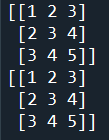程式碼內容 5.建議使用ndarray來建立矩陣 5.建議使用ndarray來建立矩陣 (1).該使用 ndarray 或是 matrix？ 根據官方文件 NumPy for Matlab users 的建議，使用 ndarray！ 因為ndarray有著以下幾個優點： A).能夠表示：向量、矩陣與張量 B).許多 NumPy 的函數輸出型別為 ndarray 而非 matrix C).ndarray 進行元素級別運算與線性代數運算時，使用的運算符號有明顯區隔 因此，建議採用 ndarray 來計算矩陣。 範例2-2：矩陣相加，相減 6.範例2-2：矩陣相加，相減 import numpy as np a = np.array([[1,2,3], [2,3,4], [3,4,5]]) #print(a) b= np.array([[1,1,1], [2,2,2], [3,3,3]]) #print(b) print('a+b=\n', a+b) print('b+a=\n', b+a) #判別兩個矩陣是否相等 print('兩個矩陣是否相等:', np.array_equal(a+b,b+a)) 成果圖示：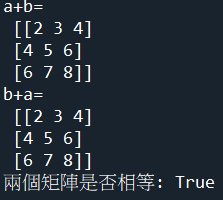程式碼內容 範例2-3：矩陣的加法與減法具有結合律 7.範例2-3：矩陣的加法與減法具有結合律 import numpy as np A = np.array([ [5, 3], [0, 1] ]) B = np.array([ [4, -1], [2, 3] ]) C = np.array([ [9, 8], [-6, 2] ]) print('(A+B)+C=\n', (A+B)+C) print('A+(B+C)=\n', A+(B+C)) #判別兩個矩陣是否相等 print('兩個矩陣是否相等:', np.array_equal((A+B)+C, A+(B+C))) 成果圖示：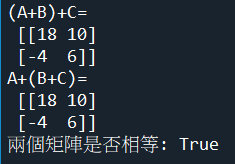程式碼內容 範例2-4：矩陣的純量相乘 8.範例2-4：矩陣的純量相乘 import numpy as np A = np.array([ [2, -3, -4, 0], [-3, 1, -1, 5], [4, 0, -6, -7] ]) k=3 print('k*A=\n',k*A) 成果圖示：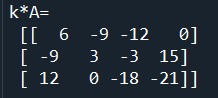程式碼內容 範例2-5：矩陣與矩陣相乘的三種方法 9.範例2-5：矩陣與矩陣相乘的三種方法： #(1)AB = A.dot(B) #(2)AB = np.dot(A,B) #(3)AB = A @ B import numpy as np A = np.array([ [4, -3, 2], [0, 1, -1], [5, 4, 0] ]) B = np.array([ [2, 2, -5], [3, 1, 0], [-1, 1, 4] ]) print('(1).AB = A.dot(B)=\n',A.dot(B)) print('(2)AB = dot(A,B)=\n',np.dot(A,B)) print('(3)AB = A @ B=\n',A @ B) 成果圖示：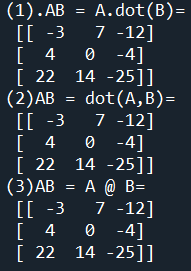程式碼內容 範例2-6：矩陣與矩陣相乘時不具有交換律 10.範例2-6：矩陣與矩陣相乘時不具有交換律 #(1).AB ≠ BA import numpy as np A = np.array([ [4, -3, 2], [0, 1, -1], [5, 4, 0] ]) B = np.array([ [2, 2, -5], [3, 1, 0], [-1, 1, 4] ]) print('(1).AB =\n',A @ B) print('(2).BA =\n',B @ A) #判別兩個矩陣是否相等 print('兩個矩陣是否相等：', np.array_equal(A @ B, B @ A)) 成果圖示：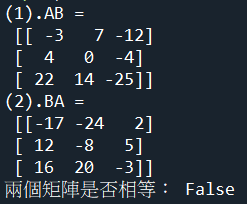程式碼內容 範例2-7：三個矩陣相乘具有結合律 11.範例2-7：三個矩陣相乘具有結合律 import numpy as np A = np.array([ [4, -1], [2, 3] ]) B = np.array([ [5, 3], [0, 1] ]) C = np.array([ [9, 8], [-6, 2] ]) print('(AB)C=\n', (A @ B) @ C) print('A(BC)=\n', A @ (B @ C)) 成果圖示：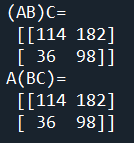程式碼內容 範例2-8：三個矩陣相乘具有分配律 12.範例2-8：三個矩陣相乘具有分配律 import numpy as np A = np.array([ [4, -1], [2, 3] ]) B = np.array([ [5, 3], [0, 1] ]) C = np.array([ [9, 8], [-6, 2] ]) print('(A(B+C)=\n', A @ (B+C)) print('AB+AC=\n', A @ B + A @ C) 成果圖示：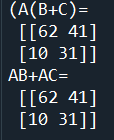程式碼內容 範例2-9：單位矩陣（Identity Matrix） 13.範例2-9：單位矩陣（Identity Matrix） #單位矩陣以大寫英文字母 I 表示：是對角線上為 1 其餘位置為 0 的矩陣 #NumPy 建立單位矩陣的方法是 np.eye() 取 I 與 Eye 諧音。 import numpy as np #建立2 x 2 的單位矩陣 a = np.eye(2) print('2 x 2 的單位矩陣=\n',a) #建立3 x 3 的單位矩陣 a = np.eye(3) print('3 x 3 的單位矩陣=\n',a) 成果圖示：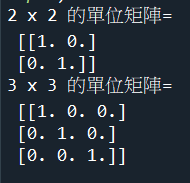程式碼內容 範例2-10：矩陣與單位矩陣相乘具有交換律 14.範例2-10：矩陣與單位矩陣相乘具有交換律 #(1).一般矩陣沒有交換律：AB ≠ BA #(2).但是單位矩陣有交換律：AI = IA import numpy as np A = np.array([ [4, -3, 2], [0, 1, -1], [5, 4, 0] ]) I = np.eye(3) print('AI=\n',A.dot(I)) print('IA=\n',I.dot(A)) print('兩個矩陣是否相等：',np.array_equal(A.dot(I),I.dot(A))) 成果圖示：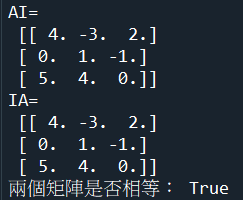程式碼內容 範例2-11：轉置矩陣 15.範例2-11：轉置矩陣 #轉置矩陣（Transpose）：將矩陣 A 中每個數字從 (m, n) 的位置轉換到 (n, m) import numpy as np A = np.array([ [1, 2], [3, 4], [5, 6] ]) B=A.T print('A矩陣=\n',A) print('A的轉置矩陣=\n',B) 成果圖示：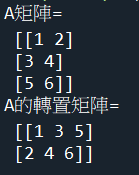程式碼內容 範例2-12：轉置矩陣具有分配律：(A+B).T = A.T + B.T 16.範例2-12：轉置矩陣具有分配律：(A+B).T = A.T + B.T import numpy as np A = np.array([ [1, 2], [3, 4], [5, 6] ]) B = np.array([ [7, 8], [9, 10], [11, 12] ]) print('(A+B).T=\n',(A+B).T) print('A.T + B.T=\n',A.T + B.T) print('兩個矩陣是否相同：', np.array_equal((A+B).T, A.T + B.T)) 成果圖示：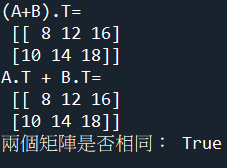程式碼內容 範例2-13：矩陣相乘的轉置具有分配律：(A*B).T = B.T * A.T 17.範例2-13：矩陣相乘的轉置具有分配律：(A*B).T = B.T * A.T #注意AB次序：(A*B).T = B.T * A.T #錯誤寫法：(A*B).T = A.T * B.T import numpy as np A = np.array([ [1, 2], [3, 4], [5, 6] ]) B = np.array([ [1, 0, 2], [4, 5, 6] ]) print('(A*B).T =\n', (A @ B).T) print('(A*B).T =\n', (B.T) @ (A.T)) 成果圖示：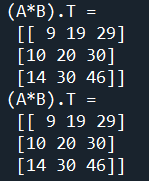程式碼內容 範例2-14：如何取出矩陣的第一列，第二列，第一欄，第二欄 18.範例2-14：如何取出矩陣的第一列，第二列，第一欄，第二欄 import numpy as np A = np.array([[1,2],[3,4]]) print('A矩陣=\n', A) print('A矩陣第一列=', A[0,:]) print('A矩陣第二列=', A[1,:]) print('A矩陣第一欄=', A[:,0]) print('A矩陣第二欄=', A[:,1]) 成果圖示：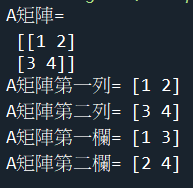程式碼內容 範例2-15：計算矩陣的維度 19.範例2-15：計算矩陣的維度 import numpy as np A = np.array([[7,1,2],[6,3,4]]) print('A矩陣=\n', A) print('矩陣A的維度=', A.shape) print('mxn矩陣A的變數m數目=', A.shape) print('mxn矩陣A的變數n數目=', A.shape) print('mxn矩陣A的輸出向量數目m=', A.shape) print('mxn矩陣A的輸入向量數目n=', A.shape) print('矩陣A的輸出變數數目的維度=',A.ndim) 成果圖示：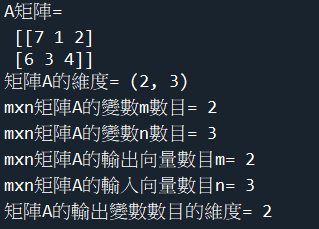程式碼內容 範例2-16：使用sympy做矩陣的乘法multiplication ，有2種方法 20.範例2-16：使用sympy做矩陣的乘法multiplication ，有2種方法： #(1)AB = A * B #(2)AB = A @ B #注意：dot是內積，不是乘法，這點與numpy不同 #(1)點積，內積=A.B = A.do(B) from sympy import * A = Matrix([ [4, -3, 2], [0, 1, -1], [5, 4, 0] ]) B = Matrix([ [2, 2, -5], [3, 1, 0], [-1, 1, 4] ]) print('(1).AB = A * B=\n',A * B) print('(3).AB = A @ B=\n',A @ B) print('注意：dot是內積，不是乘法，這點與numpy不同 = A。B = A.dot(B)') row = A @ B print('取出第一列=', row.row(0)) print('取出第一行=', row.col(0)) 成果圖示：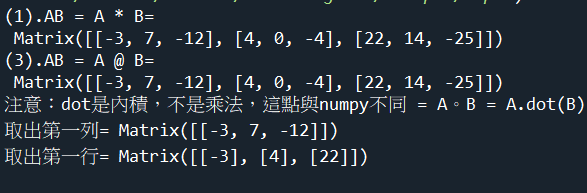程式碼內容 範例2-17：用4次多項式近似sin積分 21.範例2-17：用4次多項式近似sin積分 #已經知道5個點舒服 #x1=0, x2=0.25, x3=0.5, x4=0.75, x5=1 #y1=0, y2=𝟎.𝟎𝟗𝟖𝟎𝟏𝟕, y3=𝟎.𝟑𝟖𝟐𝟔𝟖𝟑, y4=𝟎.𝟕𝟕𝟑𝟎𝟏,y5=1 #求4次多項式 = a0 + a1×x + a2*x^2 + a3*x^3 + a4*x^4 #解出多項式的係數（a0, a1, a2, a3, a4) from sympy import * x0,x1,x2,x3,x4 = symbols('x0 x1 x2 x3 x4') #注意：M矩陣的最右邊=y=轉換後座標 A = Matrix([ [1,0,0,0,0,0], [1,0.25,0.25**2,0.25**3,0.25**4,0.098017], [1,0.5,0.5**2,0.5**3,0.5**4,0.382683], [1,0.75,0.75**2,0.75**3,0.75**4,0.77301], [1,1,1,1,1,1] ]) ans = solve_linear_system(A, x0,x1,x2,x3,x4) print('X=', ans) 成果圖示：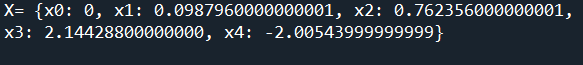程式碼內容

 chp3.反矩陣，Inverse Matrix 1.反矩陣，numpy與sympy指令的優比較 2.如何在python的sympy印出標準的數學符號 範例3-1：numpy反矩陣 範例3-2：numpy不可逆的反矩陣（行列式值=0） 範例3-3：numpy用反矩陣解力量方程式 範例3-4：numpy用反矩陣解力量方程式，先判別有唯一解 範例3-5：sympy算反矩陣 範例3-6：sympy算不可逆反矩陣 範例3-7：numpy算A的反矩陣的三次方 1.反矩陣，numpy與sympy指令的優比較 1.反矩陣，numpy與sympy指令的優比較 （1）sympy模組 ■反矩陣指令：A.inv() ■範例： from sympy import * A = Matrix([ [3, 2,-1], [1, 6,3], [2, -4,0] ]) A_inv = A.inv() print('矩陣A=\n', A_inv) （2）numpy模組 ■numpy的反矩陣指令：np.linalg.inv(A) ■範例： import numpy as np A = np.array([ [3, 2,-1], [1, 6,3], [2, -4,0] ]) A_inv = np.linalg.inv(A) print('矩陣A=\n', A_inv) 2.numpy有個專門做線性代數的模組：numpy.linalg 2.numpy有個專門做線性代數的模組：numpy.linalg NumPy - 線性代數函數 提供線性代數所需的所有功能。 此模塊中的一些重要功能如下表所述。 序號 函數及描述 1. dot： 兩個數組的點積 2. vdot： 兩個向量的點積 3. inner： 兩個數組的內積 4. matmul： 兩個數組的矩陣積 5. determinant： 數組的行列式 6. solve： 求解線性矩陣方程 7. inv： 尋找矩陣的乘法逆矩陣 numpy反矩陣指令：np.linalg.inv(x) 逆矩陣（inverse matrix）：設A是數域上的一個n階矩陣，若在相同數域上存在另一個n階矩陣B， 使得： AB=BA=E ，則我們稱B是A的逆矩陣，而A則被稱為可逆矩陣。 注：I為單位矩陣。 範例3-1：反矩陣 3.範例3-1：反矩陣 #(1).反矩陣（Inverse）：如果矩陣 M 是一個可逆矩陣（invertible），則矩陣 M #(2).算反矩陣前，要先判別是否det(M)行列式值≠0 #(3).反矩陣函數在numpy.linalg模組裡面，指令：linalg.inv import numpy as np A = np.array([ [4, -7], [2, -3] ]) A_inv = np.linalg.inv(A) print('矩陣A=\n', A) print('反矩陣=\n',A_inv) print('A*A_inv=I\n',A @ A_inv) 成果圖示：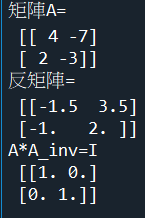程式碼內容 範例3-2：不可逆的反矩陣（行列式值=0） 4.範例3-2：不可逆的反矩陣（行列式值=0） #(1).並不是每個矩陣都具有反矩陣，以前述 2 x 2 的矩陣 M 為例，當 ad-bc 為零的時候，矩陣 M 就稱為不可逆（singular）矩陣，呼叫 np.linalg.inv() 會產生錯誤 import numpy as np A = np.array([ [8, 2], [12, 3] ]) print('矩陣A=\n', A) try: A_inv = np.linalg.inv(A) print('反矩陣=\n', A_inv) except: print('A為不可逆矩陣，沒有反矩陣') 成果圖示：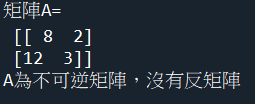程式碼內容 範例3-3：用反矩陣解力量方程式 5.範例3-3：用反矩陣解力量方程式 #x-y=1 #2x+y=6 import numpy as np A=np.array([ [1,-1], [2,1] ]) A_inv = np.linalg.inv(A) Y = np.array([ , ]) X = A_inv @ Y print('(1).反矩陣inv()，求解X=\n', X) X = np.linalg.solve(A, Y) print('(2).solve()，求解X=\n', X) 成果圖示：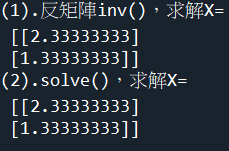程式碼內容 範例3-4：用反矩陣解力量方程式，先判別有唯一解，無解，或無限多組解 6.範例3-4：用反矩陣解力量方程式，先判別有唯一解，無解，或無限多組解 #x+y=4 #1x+3y=6 import numpy as np A = np.array([ [1,1], [3,3] ]) #注意：y矩陣，是以row為單位 Y = np.array([ , ]) AY = np.array([ [1,4], [3,6] ]) #計算行列式值 A_det = np.linalg.det(A).round() AY_det = np.linalg.det(AY).round() #先判別有唯一解，無解，或無限多組解 if A_det !=0: #有唯一解 A_inv = np.linalg.inv(A) X = A_inv @ Y print('方程式，有唯一解，X=\n', X) elif A_det ==0 and AY_det==0: print('方程式，無限多解') elif A_det ==0 and AY_det !=0: print('方程式，無解') 成果圖示：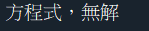程式碼內容 範例3-5：用sympy計算反矩陣 7.範例3-5：用sympy計算反矩陣 from sympy import * A = Matrix([ [3, 2,-1], [1, 6,3], [2, -4,0] ]) A_inv = A.inv() print('矩陣A=\n', A_inv) 成果圖示：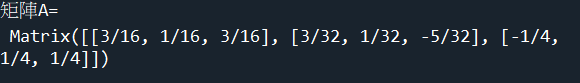程式碼內容 範例3-6：用sympy計算反矩陣，但不可逆，沒有反矩陣 8.範例3-6：用sympy計算反矩陣，但不可逆，沒有反矩陣 from sympy import * A = Matrix([ [1, 6,4], [2, 4,-1], [-1, 2,5] ]) A_inv = A.inv() print('矩陣A=\n', A_inv) 成果圖示：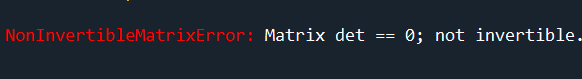程式碼內容 範例3-7：計算A的反矩陣的三次方 9.範例3-7：計算A的反矩陣的三次方 #A的 n次方：np.linalg.matrix_power(A,n) #注意：矩陣的A**3, 不是矩陣的三次方（而是每個元素的三次方而已） import numpy as np A = np.array([ [1,2], [1,3] ]) A_inv = np.linalg.inv(A) A_inv_pow3 = np.linalg.matrix_power(A_inv,3) print('反矩陣的三次方=\n',A_inv_pow3) print('反矩陣每個元素的三次方=\n',A_inv**3) 成果圖示：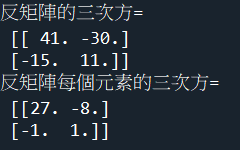程式碼內容
 chp4.行列式，determinant 1.建立行列式，numpy與sympy指令的優比較 範例4-1：計算2x2矩陣的行列式值 範例4-2：計算3x3矩陣的行列式值 範例4-3：計算4x4矩陣的行列式值 範例4-4：使用sympy計算3x3矩陣的行列式值 1.建立行列式，numpy與sympy指令的優比較 1.建立行列式，numpy與sympy指令的優比較 （1）sympy模組 ■行列式值矩陣指令：A.det() ■範例： from sympy import * A = Matrix([ [1,2,3], [-4,5,6], [7,-8,9], ]) A_det = A.det() print("A的行列式值=", A_det) （2）numpy模組 ■numpy的行列式指令：np.linalg.det(A) ■範例： import numpy as np A = np.array([ [1,2,3], [-4,5,6], [7,-8,9], ]) A_det = np.linalg.det(A) 範例4-1：計算2x2矩陣的行列式值 2.範例4-1：計算2x2矩陣的行列式值 import numpy as np A = np.array([ [5,3], [2,6] ]) A_det = np.linalg.det(A).round() print("A的行列式值=", A_det) print("A的行列式值(四捨五入)=", A_det.round()) 成果圖示：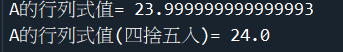程式碼內容 範例4-2：計算3x3矩陣的行列式值 2.範例4-2：計算3x3矩陣的行列式值 import numpy as np A = np.array([ [1,2,3], [-4,5,6], [7,-8,9], ]) A_det = np.linalg.det(A).round() print("A的行列式值=", A_det) print("A的行列式值(四捨五入)=", A_det.round()) 成果圖示：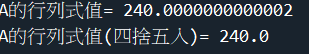程式碼內容 範例4-3：計算4x4矩陣的行列式值 3.範例4-3：計算4x4矩陣的行列式值 import numpy as np A = np.array([ [1,0,0,-1], [3,1,2,2], [1,0,-2,1], [2,0,0,1] ]) A_det = np.linalg.det(A).round() print("A的行列式值=", A_det) print("A的行列式值(四捨五入)=", A_det.round()) 成果圖示：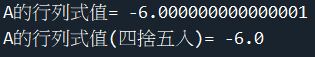程式碼內容 範例4-4：使用sympy計算3x3矩陣的行列式值 4.範例4-4：使用sympy計算3x3矩陣的行列式值 from sympy import * A = Matrix([ [1,2,3], [-4,5,6], [7,-8,9], ]) A_det = A.det() print("A的行列式值=", A_det) print("A的行列式值(四捨五入)=", A_det.round()) 成果圖示：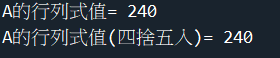程式碼內容
 chp5.求解聯立方程式 目錄 1.求解線性系統，numpy與sympy指令的優缺點 範例5-1：解聯立方程式 範例5-2：解聯立方程式 範例5-3：解聯立方程式先用行列式，判別，是否有唯一解 範例5-4：解聯立方程式先用行列式，判別，是否有唯一解 範例5-5：用反矩陣解力量方程式 範例5-6：用反矩陣解力量方程式，先判別有唯一解 範例5-7：用rank來判別，是否有唯一解 範例5-8：用rank來判別，是否有唯一解 範例5-9：用sympy模組解聯立方程式 範例5-10：sympy解聯立方程式 範例5-11：用sympy解聯立方程式，求無限多組解的通解 範例5-12：用sympy解聯立方程式，求無限多組解的通解 範例5-13：用sympy解聯立方程式，求唯一解 範例5-14：sympy把A做LU分解 1.求解線性系統，numpy與sympy指令的優缺點 1.求解線性系統，numpy與sympy指令的優缺點 （1）sympy模組 指令：solve_linear_system(M, x1,x2) 注意：M矩陣的最右邊=y=轉換後座標 from sympy import * x1,x2 = symbols('x1 x2') M = Matrix([ [1,1,4], [3,3,6] ]) ans = solve_linear_system(M, x1,x2) 優點1：可以自動處理唯一解，無解，無限多就解，不需要人工再去判別處理 優點2：無解，則自動顯示None 優點3：無限多解，則自動顯示通解（帶有x變數的通解） （2）numpy模組 指令：np.linalg.solve(A, Y) 函數可計算出矩陣形式的線性方程的解 import numpy as np A = np.array([ [4,-2], [16,-8] ]) Y = np.array([ , ]) X = np.linalg.solve(A, Y) 缺點1：只能處理唯一解，無法自動處理無解，無限多就解，需要人工再去判別處理 缺點2：若是無解，程式會出現錯誤訊息 缺點3：若是無限多組解，程式會出現錯誤訊息 缺點4：必須用行列式det(M)，或是rank（M)，人工方式判別分類，來處理『唯一解，無解，無限多解』 （3）結論：若要解線性系統，只能用sympy來求解 不要用numpy模組求解，因為無法處理『無解，無限多組的通解』 範例5-1：解聯立方程式 2.範例5-1：解聯立方程式 #x-y=1 #2x+y=6 import numpy as np A = np.array([ [1,-1], [2,1] ]) #注意：y矩陣，是以row為單位 Y = np.array([ , ]) X = np.linalg.solve(A, Y) print('X=\n', X) 成果圖示：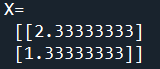程式碼內容 範例5-2：解聯立方程式 2.範例5-2：解聯立方程式 #x+y=4 #1x+3y=6 import numpy as np A = np.array([ [1,1], [3,3] ]) #注意：y矩陣，是以row為單位 Y = np.array([ , ]) try: X = np.linalg.solve(A, Y) print('X=\n', X) except: print('方程式無解') 成果圖示：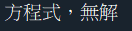程式碼內容 範例5-3：解聯立方程式先用行列式，判別，是否有唯一解，無解，或無限多解 3.範例5-3：解聯立方程式先用行列式，判別，是否有唯一解，無解，或無限多解 #若det(A)≠0，則系統有唯一解 #若det(A)＝0，且det(A|B)=0，則無限多組解 #若det(A)＝0，且det(A|B)≠0，則無解 import numpy as np A = np.array([ [1,1], [3,3] ]) #注意：y矩陣，是以row為單位 Y = np.array([ , ]) #計算行列式det(A) AY= np.array([ [1,4], [3,6] ]) A_det = np.linalg.det(A).round() AY_det = np.linalg.det(AY).round() if A_det !=0: #有唯一解 X = np.linalg.solve(A, Y) print('方程式，有唯一解，X=\n', X) elif A_det ==0 and AY_det==0: print('方程式，無限多解') elif A_det ==0 and AY_det !=0: print('方程式，無解') 成果圖示：程式碼內容 範例5-4：解聯立方程式先用行列式，判別，是否有唯一解，無解，或無限多解 4.範例5-4：解聯立方程式先用行列式，判別，是否有唯一解，無解，或無限多解 #若det(A)≠0，則系統有唯一解 #若det(A)＝0，且det(A|B)=0，則無限多組解 #若det(A)＝0，且det(A|B)≠0，則無解 import numpy as np A = np.array([ [1,-1,2], [2,-2,4], [3,-3,6] ]) #注意：y矩陣，是以row為單位 Y = np.array([ ,, ]) #計算行列式det(A) AY= np.array([ [-1,2,5], [-2,4,10], [-3,6,15], ]) A_det = np.linalg.det(A).round() AY_det = np.linalg.det(AY).round() if A_det !=0: #有唯一解 X = np.linalg.solve(A, Y) print('方程式，有唯一解，X=\n', X) elif A_det ==0 and AY_det==0: print('方程式，無限多解') elif A_det ==0 and AY_det !=0: print('方程式，無解') 成果圖示：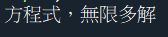程式碼內容 範例5-5：用反矩陣解力量方程式 5.範例5-5：用反矩陣解力量方程式 #x-y=1 #2x+y=6 import numpy as np A=np.array([ [1,-1], [2,1] ]) A_inv = np.linalg.inv(A) Y = np.array([ , ]) X = A_inv @ Y print('(1).反矩陣inv()，求解X=\n', X) X = np.linalg.solve(A, Y) print('(2).solve()，求解X=\n', X) 成果圖示：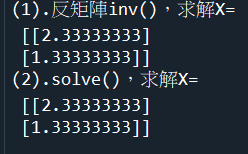程式碼內容 範例5-6：用反矩陣解力量方程式，先判別有唯一解，無解，或無限多組解 6.範例5-6：用反矩陣解力量方程式，先判別有唯一解，無解，或無限多組解 #x+y=4 #1x+3y=6 import numpy as np A = np.array([ [1,1], [3,3] ]) #注意：y矩陣，是以row為單位 Y = np.array([ , ]) AY = np.array([ [1,4], [3,6] ]) #計算行列式值 A_det = np.linalg.det(A).round() AY_det = np.linalg.det(AY).round() #先判別有唯一解，無解，或無限多組解 if A_det !=0: #有唯一解 A_inv = np.linalg.inv(A) X = A_inv @ Y print('方程式，有唯一解，X=\n', X) elif A_det ==0 and AY_det==0: print('方程式，無限多解') elif A_det ==0 and AY_det !=0: print('方程式，無解') 成果圖示：程式碼內容 範例5-7：用rank來判別，是否有唯一解，無解，或無限多解 7.範例5-7：用rank來判別，是否有唯一解，無解，或無限多解 #若rank(A)=n，表示full rank 表示有唯一解 #若 n > rank(A) 且 n > rank(AY) 表示有降階，為無限多組解 #若 n > rank(A) 且 n = rank(AY) 表示A降階，AY不降階，為無解 import numpy as np A = np.array( [ [-5, 4 ,2], [4, -5 ,2], [2, 2,-8] ]) #注意：y #注意：y矩陣，是以row為單位 Y = np.array([ ,, ]) #計算行列式det(A) AY = np.array( [ [0,-5, 4], [0,4, -5], [0,2, 2] ]) #A的維度 =n個輸入變數 n = np.shape(A) print('n=',n) A_rank = np.linalg.matrix_rank(A) print('A_rank=',A_rank) AY_rank = np.linalg.matrix_rank(AY) print('AY_rank=',AY_rank) if n == A_rank: #有唯一解 X = np.linalg.solve(A, Y) print('方程式，有唯一解，X=\n', X) elif n > A_rank and n > AY_rank: print('方程式，無限多解') elif n > A_rank and n == AY_rank: print('方程式，無解') 成果圖示：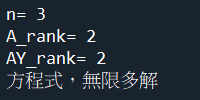程式碼內容 範例5-8：用rank來判別，是否有唯一解，無解，或無限多解 8-範例5-8：用rank來判別，是否有唯一解，無解，或無限多解 #若rank(A)=n，表示full rank 表示有唯一解 #若 n > rank(A) 且 n > rank(AY) 表示有降階，為無限多組解 #若 n > rank(A) 且 n = rank(AY) 表示A降階，AY不降階，為無解 import numpy as np A = np.array([ [1,1], [3,3] ]) #注意：y矩陣，是以row為單位 Y = np.array([ , ]) #計算行列式det(A) AY= np.array([ [1,4], [3,6] ]) #A的維度 =n個輸入變數 n = np.shape(A) print('n=',n) A_rank = np.linalg.matrix_rank(A) print('A_rank=',A_rank) AY_rank = np.linalg.matrix_rank(AY) print('AY_rank=',AY_rank) if n == A_rank: #有唯一解 X = np.linalg.solve(A, Y) print('方程式，有唯一解，X=\n', X) elif n > A_rank and n > AY_rank: print('方程式，無限多解') elif n > A_rank and n == AY_rank: print('方程式，無解') 成果圖示：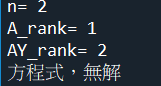程式碼內容 範例5-9：用sympy模組解聯立方程式 9.範例5-9：用sympy模組解聯立方程式 #x-y=1 #2x+y=6 from sympy import * x1,x2 = symbols('x1 x2') #注意：M矩陣的最右邊=y=轉換後座標 A = Matrix([ [1,-1,1,], [2,1,6] ]) ans = solve_linear_system(A, x1, x2) print('X=', ans) 成果圖示：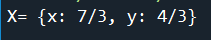程式碼內容 範例5-10：用sympy解聯立方程式 10.範例5-10：用sympy解聯立方程式 #x+y=4 #1x+3y=6 from sympy import * x1,x2 = symbols('x1 x2') #注意：M矩陣的最右邊=y=轉換後座標 A = Matrix([ [1,1,4], [3,3,6] ]) ans = solve_linear_system(A, x1, x2) print('X=', ans) 成果圖示：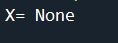程式碼內容 範例5-11：用sympy解聯立方程式，求無限多組解的通解 11.範例5-11：用sympy解聯立方程式，求無限多組解的通解 from sympy import * x1,x2,x3 = symbols('x1 x2 x3') #注意：M矩陣的最右邊=y=轉換後座標 A = Matrix([ [1,-1,2,5], [2,-2,4,10], [3,-3,6,15] ]) ans = solve_linear_system(A, x1, x2, x3) print('X=', ans) 成果圖示：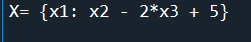程式碼內容 範例5-12：用sympy解聯立方程式，求無限多組解的通解 12.範例5-12：用sympy解聯立方程式，求無限多組解的通解 from sympy import * x1,x2,x3,x4,x5,x6 = symbols('x1 x2 x3 x4 x5 x6') #注意：M矩陣的最右邊=y=轉換後座標 A = Matrix([ [1,3,-2,0,2,0,0], [2,6,-5,-2,4,-3,-1], [0,0,5,10,0,15,5], [2,6,0,8,4,18,6] ]) ans = solve_linear_system(A, x1, x2, x3, x4,x5,x6) print('X=', ans) 成果圖示：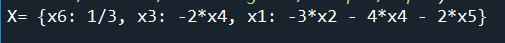程式碼內容 範例5-13：用sympy解聯立方程式，求唯一解 13.範例5-13：用sympy解聯立方程式，求唯一解 from sympy import * x1,x2,x3,x4 = symbols('x1 x2 x3 x4') #注意：M矩陣的最右邊=y=轉換後座標 A = Matrix([ [1,3,7,2,5], [0,1,2,-4,1], [0,0,1,6,9], [1,0,0,0,1] ]) ans = solve_linear_system(A, x1, x2, x3, x4) print('X=', ans) 成果圖示：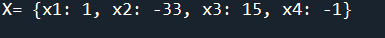程式碼內容 範例5-14：sympy把A做LU分解 13.範例5-14：sympy把A做LU分解 from sympy import * x1,x2 = symbols('x1 x2') A = Matrix([ [2,6,2], [-3,-8,0], [4,9,2] ]) L, U, _ = A.LUdecomposition() print(L) print(U) 成果圖示：程式碼內容
 chp6.向量 範例6-1：若向量 a=(3,−1) ，b=(2,1) ，求二者如何组合成向量c 範例6-2：若向量 a=(3,−1) 係數乘法，求3a 範例6-3：求向量的點積:a.dot(b) 範例6-4：求向量的外積： np.cross(a,b) 範例6-1：若向量 a=(3,−1) ，b=(2,1) ，求二者如何组合成向量c 1.範例6-1：若向量 a=(3,−1) ，b=(2,1) ，求二者如何组合成向量c import numpy as np #比較：矩陣與向量 #矩陣：np.array([ [1,-1], [2,1] ]) #向量：np.array([3,-1]) a = np.array([3,-1]) b = np.array([2,1]) c = a+b print('c=a+b=\n', c) 成果圖示：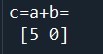程式碼內容 範例6-2：若向量 a=(3,−1) 係數乘法，求3a 2.範例6-2：若向量 a=(3,−1) 係數乘法，求3a #比較：矩陣與向量 #矩陣：np.array([ [1,-1], [2,1] ]) #向量：np.array([3,-1]) import numpy as np a = np.array([3,-1]) c = 3*a print('c=3a=\n', c) 成果圖示：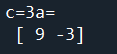程式碼內容 範例6-3：求向量的點積:a.dot(b) 3.範例6-3：求向量的點積:a.dot(b) #從幾何的角度來說，向量的點積與向量間夾角 θ 的餘弦有關： a⃗ ⋅b⃗ =∥a⃗ ∥*∥b⃗ ∥*cosθ #故向量點積=向量的點積其實反映了向量 a⃗ 在向量 b⃗ 上的 投影 #向量 a=(3,5,2)， b=(1,4,7) import numpy as np a = np.array([3,5,2]) b = np.array([1,4,7]) print('向量點積有三種指令：') print('a.dot(b)=', a.dot(b)) print('np.dot(a,b)=', np.dot(a,b)) print('a @ b=', a @ b) 成果圖示：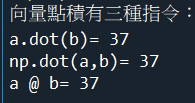程式碼內容 範例6-4：求向量的外積： np.cross(a,b) 4.範例6-4：求向量的外積： np.cross(a,b) #向量外積=叉乘、向量積、叉積 #外積的一個重要作用：是可以得到一個和 a⃗ 、b⃗ 兩個原向量垂直正交的新向量 c⃗ ，且可以通過右手法則來確定新向量的方向 #向量的外積，反映了向量 a⃗ 與向量 b⃗ 的垂直正交程度：當兩向量平行時，sinθ 的值為0，外積的值為0，正交程度最小。 #當兩向量垂直正交時：sinθ 的值為1，外積值最大，正交程度最大 #向量 a=(3,5,2)， b=(1,4,7) import numpy as np a = np.array([3,5,2]) b = np.array([1,4,7]) print('外積=np.croos(a,b)=', np.cross(a,b)) 成果圖示：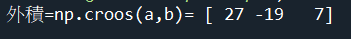程式碼內容
 chp7.張量tensor 1.張量tensor的定義有兩種 2.python裡面的張量 3.張量是矩陣的擴展與延伸 4.Tensor的對像有三個屬性 6.張量的階數有時候也稱為維度，或者軸 範例7-1：張量tensor 8.python裡面的張量tensor，向量vector，純量scalar 範例7-2：python裡面的張量tensor，向量vector，純量scalar 1.張量tensor的定義有兩種 1.張量tensor的定義有兩種 (1)用多維度陣列的數組來做解釋（X) 這些是純粹用陣列數字的方式來解釋張量tensor 但是這不是張量的原始定義 (2).張量是描述幾何geometry坐標的方法 https://www.youtube.com/watch?v=TvxmkZmBa-k 第2：00部分解釋 https://www.youtube.com/watch?v=sNrJ3l6mK3Y 5：00的解釋 https://www.itread01.com/content/1547313962.html 2.python裡面的張量 2.python裡面的張量 # a rank 0 tensor; this is a scalar with shape [] 3 # a rank 1 tensor; this is a vector with shape  [1. ,2., 3.] # a rank 2 tensor; a matrix with shape [2, 3] [[1., 2., 3.], [4., 5., 6.]] # a rank 3 tensor with shape [2, 1, 3] 常說的張量就是3階張量 [[[1., 2., 3.]], [[7., 8., 9.]]] 3.張量是矩陣的擴展與延伸 3.張量是矩陣的擴展與延伸 標量：只有大小 向量：有大小和方向 矩陣：由行列兩個向量組成 3階張量：由行列高三個向量組成 n階張量：由N個互相垂直的向量組成 4.Tensor的對像有三個屬性 4.Tensor的對像有三個屬性： （1）rank：number of dimensions （2）shape： number of rows and columns （3）type：data type of tensor's elements 6.張量的階數有時候也稱為維度，或者軸 6.張量的階數有時候也稱為維度，或者軸 譬如一個矩陣[[1,2],[3,4]]，是一個2階張量，有兩個維度或軸， 沿著第0個軸（為了與python的計數方式一致）你看到的是[1,2]，[3,4]兩個向量， 沿著第1個軸你看到的是[1,3]，[2,4]兩個向量。 範例7-1：張量tensor 7.範例7-1：下面的程式碼可以幫助理解張量tensor“沿着某個軸”是什麼意思 import numpy as np a = np.array([[1,2],[3,4]]) sum0 = np.sum(a, axis=0) sum1 = np.sum(a, axis=1) print('矩陣a=\n', a) #2 階張量，有兩個維度或軸，沿著第 0 個軸= [1,2]、[3,4] 兩個向量 print('2 階張量，有兩個維度或軸，沿著第0個軸==', a[0,:],a[1,:]) print('2 階張量，有兩個維度或軸，沿著第1個軸==', a[:,0],a[:,1]) #2 階張量，有兩個維度或軸，沿著第 0 個軸= [1,2]、[3,4] 兩個向量 print('2 階張量，有兩個維度或軸，沿著第 0 個軸，總和=', sum0) #2 階張量，有兩個維度或軸，沿著第 1 個軸= [1,3]、[2,4] 兩個向量 print('2 階張量，有兩個維度或軸，沿著第 1 個軸，總和=', sum1) 成果圖示：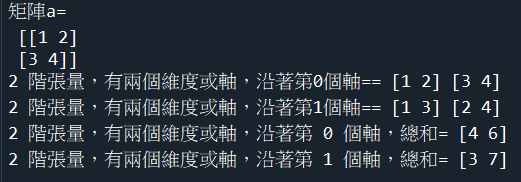程式碼內容 8.python裡面的張量tensor，向量vector，純量scalar 8.python裡面的張量tensor，向量vector，純量scalar (1).定義：python裡面的張量tensor，向量vector，純量scalar，是根據多維陣列的數組型態來定義的。 這些定義只是方便python的計算方便，但並不是張量的原始物理意義。 張量的原始物理意義：乃是在線性代數(linear algebra)描述不同坐標系統的某個幾何物體的方式， (2)從陣列的數組角度來定義 圖示：圖示：範例7-2：python裡面的張量tensor，向量vector，純量scalar 9.範例7-2：python裡面的張量tensor，向量vector，純量scalar import numpy as np #1- 純量scalar (rank=0) a1 = 5 print('純量scalar=', a1) #2-python 向量vector（rank=1） a2 = [1,5,7] print('python向量vector(不適合做矩陣運算)=', a2) #3-numpy向量vector（rank=1） a3 = np.array([5,6,7]) print('numpy向量vector(適合做矩陣運算)=', a3) #4-nump矩陣Matrix（rank=2） a4 = np.array([[5,6,7],[2,8,9]]) print('nump矩陣Matrix（rank=2）=\n', a4) #5-nump張量tensor（rank=3） a5 = np.array([[[5,6,7],[2,8,9]],[[15,16,17],[12,18,19]]]) print('nump張量tensor（rank=3）=\n', a5) 成果圖示：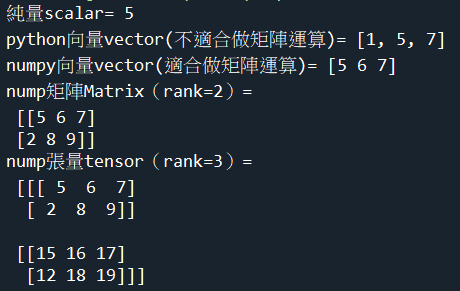程式碼內容
 chp8.秩rank，跡tracer 1.rank：numpy與sympy指令的優比較 範例8-1：求矩陣的rank根 範例8-2：求矩陣的跡tracer 範例8-3：用sympy求矩陣的rank 1.rank：numpy與sympy指令的優比較 1.rank：numpy與sympy指令的優比較 （1）sympy模組 ■rank指令：A.rank() ■範例： from sympy import * A = Matrix([ [1,2,3], [-4,5,6], [7,-8,9], ]) A_rank = rank() print("A的rank=", A_rank) （2）numpy模組 ■rank指令：np.linalg.matrix_rank(A) ■範例： import numpy as np A = np.array([ [1,2,3], [-4,5,6], [7,-8,9], ]) A_rank = np.linalg.matrix_rank(A) print("A的rank=", A_rank) 2.範例8-1：求矩陣的rank 2.範例8-1：求矩陣的rank #線性代數的rank=秩 #注意：在 Numpy 中的秩和線性代數里的秩是不同的概念。在NumPy中維度（dimensions）叫做軸（axes） #矩陣A的維度=', A.shape #矩陣A的變數n數目=', A.shape import numpy as np A = np.array([ [-1,2,0,4,5,-3], [3,-7,2,0,1,4], [2,-5,2,4,6,1], [4,-9,2,-4,-4,7] ]) rankA = np.linalg.matrix_rank(A) print('矩陣A的rank=',rankA) print('矩陣A的維度=',A.ndim) print('矩陣A的維度=', A.shape) print('矩陣A的變數n數目=', A.shape) 成果圖示：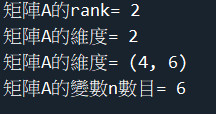程式碼內容 範例8-2：求矩陣的跡tracer 2.範例8-2：求矩陣的跡tracer #跡tracer=對角線值相乘指令=np.trace(A) import numpy as np A = np.array([ [-1,2,7,0], [3,5,-8,4], [1,2,7,-3], [4,-2,1,0] ]) print('A的跡tracer=',np.trace(A)) 成果圖示：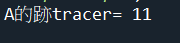程式碼內容 範例8-3：用sympy求矩陣的rank 3.範例8-3：用sympy求矩陣的rank #線性代數的rank=秩 = A.rank() #矩陣A的變數n數目=', A.shape from sympy import * A = Matrix([ [-1,2,0,4,5,-3], [3,-7,2,0,1,4], [2,-5,2,4,6,1], [4,-9,2,-4,-4,7] ]) rankA = A.rank() print('矩陣A的rank=',rankA) print('矩陣A的變數n數目=', A.shape) 成果圖示：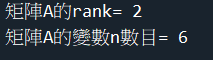程式碼內容
 chp9.線性獨立，線性相依 1.判別向量之間是線性獨立，或線性相依的方法，有3種 範例9-1：判別幾個向量之間，是線性獨立否？ 範例9-2：判別幾個向量之間，是線性獨立否？ 範例9-3：判別幾個多項式之間，是線性獨立否？ 範例9-4：判別幾個向量之間，是線性獨立否？ 範例9-5：判別幾個多項式之間，是線性獨立否？ 範例9-6：判別幾個向量可否成為R2的基底向量？ 範例9-7：判別幾個向量可否成為R2的基底向量？ 範例9-8：判別幾個向量可否成為R2的基底向量？ 範例9-9：用sympy判別三個向量之間，是線性獨立否？ 範例9-10：用sympy判別三個向量之間，是線性獨立否？ 1.判別向量之間是線性獨立，或線性相依的方法，有3種 1.判別向量之間是線性獨立，或線性相依的方法，有3種： 證明向量v1=(1,2,1),v2=(2,9,0)和v3=(3,3,4)可為 R3 的基底 (1).判別向量是否為線性獨立，方法1： 三個向量必須是線性獨立， 故C1v1+C2v2 +C3v3=0，C除了0外無解 解 C 的聯立方程式。 若存在c向量合成𝟎（C有解），則線性相依 若不存在C向量合成𝟎（C無解），則線性獨立 (2).判別向量是否為線性獨立，方法2： 先計算系統的輸入變數數量n 再計算系統矩陣的秩rank 若n > rank，則表示系統有降階，表示向量之間有線性相依 若n = rank，則表示full rank，表示系統沒有降階，表示向量之間有線性獨立 (3).方法3：用sympy的化簡成梯形矩陣指令 會傳回線性獨立的行向量index 指令：A_reduced_form, inds = A.rref() inds = 記錄線性獨立的行向量index（culumn index) #注意：若是三個向量，就會有三個輸入變數k1,k2,k3 #所以k1的行向量=v1=(1,-2,3) 範例： from sympy import * A = Matrix( [ [1, 5 ,3], [-2, 6 ,2], [3, -1 ,1] ]) A_reduced_form, inds = A.rref() print('簡化後的梯形Am=', A_reduced_form) n = A.shape n_independent = len(inds) if n == n_independent: print('三個向量彼此線性獨立') else: print('三個向量彼此線性相依') 範例9-1：判別幾個向量之間，是線性獨立，或線性相依？ 2.範例9-1：判別幾個向量之間，是線性獨立，或線性相依？ #三個向量：v1=(1,–2,3),v2=(5,6,–1),v3=(3,2,1) #判別向量是否為線性獨立，方法2： #先計算系統的輸入變數數量n #再計算系統矩陣的秩rank #若n > rank，則表示系統有降階，表示向量之間有線性相依 #若n = rank，則表示full rank，表示系統沒有降階，表示向量之間有線性獨立 import numpy as np A = np.array( [ [1, -2 ,3], [5, 6 ,-1], [3, 2 ,1] ]) #full rank就是linear independence線性獨立 # n =輸入向量數目 n = np.shape(A) print('A的輸入變數數目m=', n) A_rank = np.linalg.matrix_rank(A) print('rank=',A_rank) if n > A_rank: print('有降階，表示向量是線性相依') else: print('沒有降階，full rank， 表示向量是線性獨立') 成果圖示：程式碼內容 範例9-2：判別幾個向量之間，是線性獨立，或線性相依？ 2.範例9-2：判別幾個向量之間，是線性獨立，或線性相依？ #三個向量：v1=(1,2,2,–1), v2=(4,9,9,–4), v3=(5,8,9,–5) #判別幾個向量之間，是線性獨立，或線性相依？ import numpy as np A = np.array( [ [1, 2 ,2,-1], [4, 9 ,9,-4], [5, 8 ,9,-5] ]) #full rank就是linear independence線性獨立 # m =輸入向量數目 m = np.shape(A) print('A的輸入變數數目m=三個向量，三個k=3=', m) A_rank = np.linalg.matrix_rank(A) print('rank=',A_rank) if m > A_rank: print('有降階，表示向量是線性相依') else: print('沒有降階，full rank， 表示向量是線性獨立') 成果圖示：程式碼內容 範例9-3：判別幾個多項式之間，是線性獨立，或線性相依？ 3.範例9-3：判別幾個多項式之間，是線性獨立，或線性相依？ #三個多項式：p1=1-x, p2=5+3x-2x**2, p3=1+3x-x**2 #判別幾個多項式向量之間，是線性獨立，或線性相依？ #三個向量：v1=(1,–1,0),v2=(5,3,–2),v3=(1,3,-1) import numpy as np A = np.array( [ [1, -1 ,0], [5, 3 ,-2], [1, 3 ,-1] ]) #full rank就是linear independence線性獨立 # n =輸入向量數目 n = np.shape(A) print('A的輸入變數數目m=', n) A_rank = np.linalg.matrix_rank(A) print('rank=',A_rank) if n > A_rank: print('有降階，表示向量是線性相依') else: print('沒有降階，full rank， 表示向量是線性獨立') 成果圖示：程式碼內容 範例9-4：判別幾個向量之間，是線性獨立，或線性相依？ 4.範例9-4：判別幾個向量之間，是線性獨立，或線性相依？ #三個向量：v1=(1,–2,3),v2=(5,6,–1),v3=(3,2,1) import numpy as np A = np.array( [ [1, 2 ,1], [2, 9 ,0], [3, 3 ,4] ]) #full rank就是linear independence線性獨立 # n =輸入向量數目 n = np.shape(A) print('A的輸入變數數目m=', n) A_rank = np.linalg.matrix_rank(A) print('rank=',A_rank) if n > A_rank: print('有降階，表示向量是線性相依') else: print('沒有降階，full rank， 表示向量是線性獨立') 成果圖示：程式碼內容 範例9-5：判別幾個多項式之間，是線性獨立，或線性相依？ 5.範例9-5：判別幾個多項式之間，是線性獨立，或線性相依？ #三個多項式：p1=1-x**2, p2=2-x**2, p3=x**3 #判別幾個多項式向量之間，是線性獨立，或線性相依？ #三個向量：v1=(1,0,–1,0),v2=(2,0,-1,0),v3=(0,0,0,1) import numpy as np A = np.array( [ [1, 0 ,-1,0], [2, 0 ,-1,0], [0, 0 ,0,1] ]) #full rank就是linear independence線性獨立 # m =輸入向量數目 m = np.shape(A) print('A的輸入變數數目m=三個多項式=', m) A_rank = np.linalg.matrix_rank(A) print('rank=',A_rank) if m > A_rank: print('有降階，表示向量是線性相依') else: print('沒有降階，full rank， 表示多項式向量是線性獨立') 成果圖示：程式碼內容 範例9-6：判別幾個向量可否成為R2的基底向量？ 6.範例9-6：判別幾個向量可否成為R2的基底向量？ #二個向量：v1=(–3,7),v2=(5,5) #判別向量之間，是線性獨立，或線性相依？ import numpy as np A = np.array( [ [-3, 7], [5,5] ]) #full rank就是linear independence線性獨立 # n =輸入向量數目 n = np.shape(A) print('A的輸入變數數目m=', n) A_rank = np.linalg.matrix_rank(A) print('rank=',A_rank) if n > A_rank: print('有降階，表示向量是線性相依') else: print('沒有降階，full rank， 表示向量是線性獨立') 成果圖示：程式碼內容 範例9-7：判別幾個向量可否成為R2的基底向量？ 7.範例9-7：判別幾個向量可否成為R2的基底向量？ #二個向量：v1=(2,0,-1),v2=(4,0,7),v3=(-1,1,4) #注意：因為三個向量，所以三個輸入變數k1,k2,k3 #判別向量之間，是線性獨立，或線性相依？ import numpy as np A = np.array( [ [2, 0 ,-1], [2, 0 ,7], [-1,1 ,4] ]) #full rank就是linear independence線性獨立 # n =輸入向量數目 n = np.shape(A) print('A的輸入變數數目m=', n) A_rank = np.linalg.matrix_rank(A) print('rank=',A_rank) if n > A_rank: print('有降階，表示向量是線性相依') else: print('沒有降階，full rank， 表示向量是線性獨立') 成果圖示：程式碼內容 範例9-8：用sympy判別三個向量之間，是線性獨立，或線性相依？ 8.範例9-8：用sympy判別三個向量之間，是線性獨立，或線性相依？ #三個向量：v1=(1,–2,3),v2=(5,6,–1),v3=(3,2,1) #注意：因為三個向量，所以三個輸入變數k1,k2,k3 #所以k1的行向量=v1=(1,-2,3) #判別向量是否為線性獨立，方法3：A_reduced_form, inds = A.rref() #inds = 記錄線性獨立的行向量index（culumn index) from sympy import * A = Matrix( [ [1, 5 ,3], [-2, 6 ,2], [3, -1 ,1] ]) A_reduced_form, inds = A.rref() print('簡化後的梯形Am=', A_reduced_form) n = A.shape n_independent = len(inds) if n == n_independent: print('三個向量彼此線性獨立') else: print('三個向量彼此線性相依') print('彼此線性獨立的行數m=', inds) c1 = inds c2 = inds print('印出線性獨立的向量=', A[:,c1]) print('印出線性獨立的向量=', A[:,c2]) 成果圖示：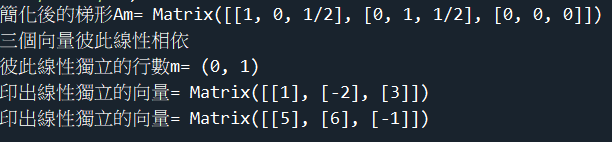程式碼內容 範例9-9：用sympy判別三個向量之間，是線性獨立，或線性相依？ 9.範例9-9：用sympy判別三個向量之間，是線性獨立，或線性相依？ #三個向量：v1=(1,2,–2,-1),v2=(4,9,9,–4),v3=(5,8,9,-5) #注意：因為三個向量，所以三個輸入變數k1,k2,k3 #所以k1的行向量=v1=(1,2,–2,-1) #判別向量是否為線性獨立，方法3：A_reduced_form, inds = A.rref() #inds = 記錄線性獨立的行向量index（culumn index) from sympy import * A = Matrix( [ [1, 4 ,8], [2, 9 ,8], [-2, 9 ,9], [-1, -4 ,-5] ]) A_reduced_form, inds = A.rref() print('簡化後的梯形Am=', A_reduced_form) n = A.shape n_independent = len(inds) if n == n_independent: print('三個向量彼此線性獨立') else: print('三個向量彼此線性相依') print('彼此線性獨立的行數m=', inds) c1 = inds c2 = inds c3 = inds print('印出線性獨立的向量=', A[:,c1]) print('印出線性獨立的向量=', A[:,c2]) print('印出線性獨立的向量=', A[:,c3]) 成果圖示：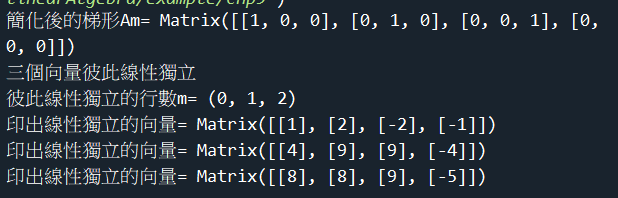程式碼內容 範例9-10：用sympy判別三個多項式向量之間，是線性獨立，或線性相依？ 10.範例9-10：用sympy判別三個多項式向量之間，是線性獨立，或線性相依？ #三個多項式：p1=1-x, p2=5+3x-2x^2, p3=1+3x-x^2 #注意：因為三個多項式向量，所以三個輸入變數k1,k2,k3 #所以k1的行向量=v2=(5,3,–2) #v1=(1,–1,0),v2=(5,3,–2),v3=(1,3,–1) #判別向量是否為線性獨立，方法3：A_reduced_form, inds = A.rref() #inds = 記錄線性獨立的行向量index（culumn index) from sympy import * A = Matrix( [ [1, 5 ,1], [-1, 3 ,3], [0, -2,-1] ]) A_reduced_form, inds = A.rref() print('簡化後的梯形Am=', A_reduced_form) n = A.shape n_independent = len(inds) if n == n_independent: print('三個向量彼此線性獨立') else: print('三個向量彼此線性相依') print('彼此線性獨立的行數m=', inds) c1 = inds c2 = inds print('印出線性獨立的向量=', A[:,c1]) print('印出線性獨立的向量=', A[:,c2]) 成果圖示：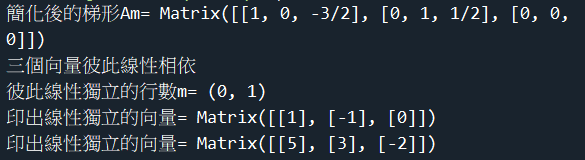程式碼內容
 chp10.特徵向量eigenVector，特徵值eigenValue 1.建立特徵向量，numpy與sympy指令的優比較 2.特徵向量，特徵值 範例10-1：計算特徵值，特徵向量 範例10-2：計算特徵值，特徵向量 範例10-3：計算3x3矩陣的特徵值，特徵向量 範例10-4：計算特特徵空間的結構（基底向量） 範例10-5：計算特特徵空間的結構（基底向量） 範例10-6：計算特特徵空間的結構 範例10-7：計算A的13次方 範例10-8：用sympy計算特徵值，特徵向量 1.建立特徵向量，numpy與sympy指令的優比較 1.建立特徵向量，numpy與sympy指令的優比較 （1）sympy模組 ■特徵向量指令：M.eigenvects() ■特徵值指令：M.eigenvals() ■範例： from sympy import * A = Matrix([ [3,0], [8,-1] ]) eigval = A.eigenvals() eigvec = A.eigenvects() print('特徵值=', eigval) print('特徵向量=', eigvec) print('第一個特徵值=', eigvec,'第一個特徵向量=',eigvec) print('第二個特徵值=', eigvec,'第二個特徵向量=',eigvec) （2）numpy模組 ■numpy計算特徵值指令：numpy.linalg.eig (1).numpy.linalg.eigvals方法：求解一個方陣的特徵值 (2).numpy.linalg.eig 方法：求解方阵的特征值和特征向量 ■範例： import numpy as np A = np.array([ [3,0], [8,-1] ]) eigval = np.linalg.eigvals(A) eigvec = np.linalg.eig(A) print('特徵值=', eigval) print('特徵向量=', eigvec) print('特徵值=', eigvec) print('特徵向量=', eigvec) print('第1個特徵值=', eigval,'第1個特徵向量=',eigvec[:,0]) print('第2個特徵值=', eigval,'第2個特徵向量=',eigvec[:,1]/eigvec[:,1]) 2.特徵向量，特徵值 2.特徵向量，特徵值 A𝒗 =λ𝒗 ⃑ 𝒗：特徵向量，Eigenvectors λ：特徵值，Eigenvalues (1).A𝒗 ⃑轉換後的向量p = 原本的向量𝒗 的縮放λ倍 (2).也就是v經過A轉換後的向量，就是v它自己，只是縮放λ倍 範例10-1：計算特徵值，特徵向量 3.範例10-1：計算特徵值，特徵向量 #(1).numpy.linalg.eigvals方法：求解一個方陣的特徵值 #(2).numpy.linalg.eig 方法：求解方阵的特徵值和特徵向量 import numpy as np A = np.array([ [3,0], [8,-1] ]) eigval = np.linalg.eigvals(A) eigvec = np.linalg.eig(A) print('特徵值=', eigval) print('特徵向量=', eigvec) print('特徵值=', eigvec) print('特徵向量=', eigvec) print('第1個特徵值=', eigval,'第1個特徵向量=',eigvec[:,0]) print('第2個特徵值=', eigval,'第2個特徵向量=',eigvec[:,1]/eigvec[:,1]) 成果圖示：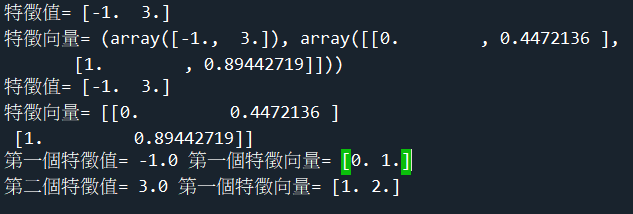程式碼內容 範例10-2：計算特徵值，特徵向量 4.範例10-2：計算特徵值，特徵向量 #(1).numpy.linalg.eigvals方法：求解一個方陣的特徵值 #(2).numpy.linalg.eig 方法：求解方阵的特徵值和特徵向量 import numpy as np A = np.array([ [3,1], [0,2] ]) eigval = np.linalg.eigvals(A) eigvec = np.linalg.eig(A) print('特徵值=', eigval) print('特徵向量=', eigvec) print('特徵值=', eigvec) print('特徵向量=', eigvec) print('第1個特徵值=', eigval,'第1個特徵向量=',eigvec[:,0]) print('第2個特徵值=', eigval,'第2個特徵向量=',eigvec[:,1]/eigvec[:,1]) 成果圖示：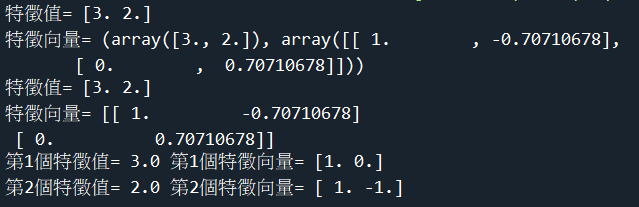程式碼內容 範例10-3：計算3x3矩陣的特徵值，特徵向量 5.範例10-3：計算3x3矩陣的特徵值，特徵向量 #(1).numpy.linalg.eigvals方法：求解一個方陣的特徵值 #(2).numpy.linalg.eig 方法：求解方阵的特徵值和特徵向量 import numpy as np A = np.array([ [0,1,0], [0,0,1], [4,-17,8] ]) eigval = np.linalg.eigvals(A) eigvec = np.linalg.eig(A) print('特徵值=', eigval) print('特徵向量=', eigvec) print('特徵值=', eigvec) print('特徵向量=', eigvec) print('第1個特徵值=', eigval,'第1個特徵向量=',eigvec[:,0]/eigvec[:,0]) print('第2個特徵值=', eigval,'第2個特徵向量=',eigvec[:,1]/eigvec[:,1]) print('第3個特徵值=', eigval,'第3個特徵向量=',eigvec[:,2]/eigvec[:,2]) 成果圖示：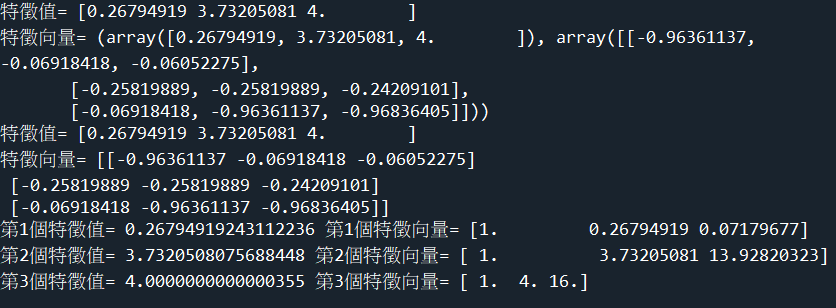程式碼內容 範例10-4：計算特特徵空間的結構（基底向量） 6.範例10-4：計算特特徵空間的結構（基底向量） #(1).numpy.linalg.eigvals方法：求解一個方陣的特徵值 #(2).numpy.linalg.eig 方法：求解方阵的特徵值和特徵向量 import numpy as np A = np.array([ [0,0,-2], [1,2,1], [1,0,3] ]) eigval = np.linalg.eigvals(A) eigvec = np.linalg.eig(A) print('特徵值=', eigval) print('特徵向量=', eigvec) print('特徵值=', eigvec) print('特徵向量=', eigvec) print('第1個特徵值=', eigval,'第1個特徵向量=',eigvec[:,0]) print('第2個特徵值=', eigval,'第2個特徵向量=',eigvec[:,1]/eigvec[:,1]) print('第3個特徵值=', eigval,'第3個特徵向量=',eigvec[:,2]/eigvec[:,2]) 成果圖示：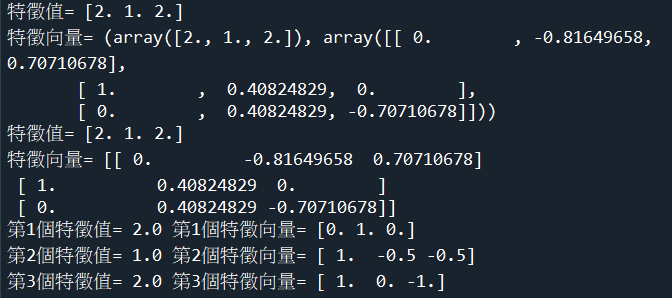程式碼內容 範例10-5：計算特特徵空間的結構（基底向量） 7.範例10-5：計算特特徵空間的結構（基底向量） #(1).numpy.linalg.eigvals方法：求解一個方陣的特徵值 #(2).numpy.linalg.eig 方法：求解方阵的特徵值和特徵向量 import numpy as np A = np.array([ [2,1,0], [0,2,0], [0,0,2] ]) #eigval = np.linalg.eigvals(A) eigval, eigvec = np.linalg.eig(A) print('特徵值=', eigval) print('特徵向量=', eigvec) print('第1個特徵值=', eigval,'第1個特徵向量=',eigvec[:,0]) print('第2個特徵值=', eigval,'第2個特徵向量=',(eigvec[:,1]/eigvec[:,1]).round()) print('第3個特徵值=', eigval,'第3個特徵向量=',eigvec[:,2]) 成果圖示：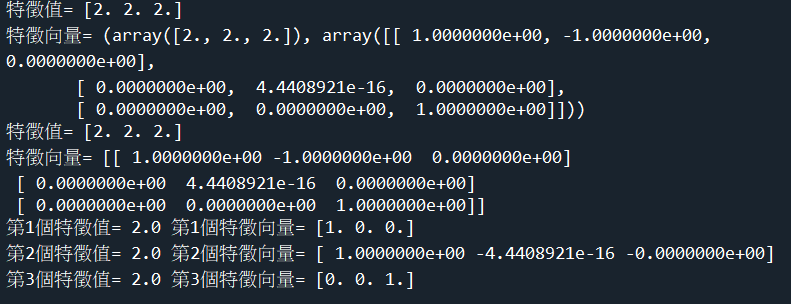程式碼內容 範例10-6：計算特特徵空間的結構（基底向量） 8.範例10-6：計算特特徵空間的結構（基底向量） #(1).numpy.linalg.eigvals方法：求解一個方陣的特徵值 #(2).numpy.linalg.eig 方法：求解方阵的特徵值和特徵向量 import numpy as np A = np.array([ [5,4,2], [4,5,2], [2,2,2] ]) #eigval = np.linalg.eigvals(A) eigval, eigvec = np.linalg.eig(A) print('特徵值=', eigval) print('特徵向量=', eigvec) print('第1個特徵值=', eigval,'第1個特徵向量=',eigvec[:,0]/eigvec[:,0]) print('第2個特徵值=', eigval,'第2個特徵向量=',eigvec[:,1]/eigvec[:,1]) print('第3個特徵值=', eigval,'第3個特徵向量=',eigvec[:,2]/eigvec[:,2]) 成果圖示：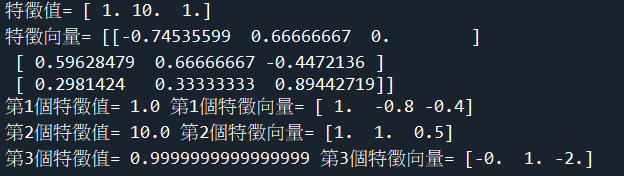程式碼內容 範例10-7：計算A的13次方 9.範例10-7：計算A的13次方 #(1).A的 n次方：np.linalg.matrix_power(A,n) #(2).A的 3次方= A @ A @ A import numpy as np A = np.array([ [0,0,-2], [1,2,1], [1,0,3] ]) A_power = np.linalg.matrix_power(A,13) print('A的 13次方=\n',A_power) print('A的 3次方=\n',A @ A @ A) 成果圖示：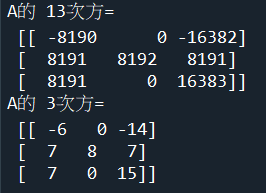程式碼內容 範例10-8：用sympy計算特徵值，特徵向量 10.範例10-8：用sympy計算特徵值，特徵向量 #■特徵向量指令：M.eigenvects() #■特徵值指令：M.eigenvals() from sympy import * A = Matrix([ [3,0], [8,-1] ]) eigval = A.eigenvals() eigvec = A.eigenvects() print('特徵值=', eigval) print('特徵向量=', eigvec) print('第一個特徵值=', eigvec,'第一個特徵向量=',eigvec) print('第二個特徵值=', eigvec,'第二個特徵向量=',eigvec) 成果圖示：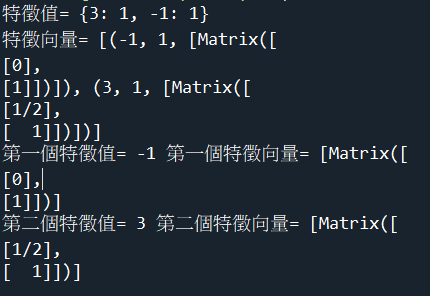程式碼內容
 chp11 線性轉換 1.線性轉換=線性映射 範例11-1：求 x=(1,1)逆時針旋轉30°的像 範例11-2：求 x=(2,3)，以原點逆時針旋轉90°，再施加水平向1剪力變換shear的像 範例11-3：求點 (3,4,5) 對xz平面鏡射後的像 範例11-4：求點(-3,1)對x軸的正交投影 範例11-5：求點(-2,1,2)，以y軸為中心旋轉45°旋轉後的像 < 1.傅立葉級數的應用 1.線性轉換=線性映射 公式：W=TA x= [T]x = 0 範例11-1：求 x=(1,1)逆時針旋轉30°的像 2.範例11-1：求 x=(1,1)逆時針旋轉30°的像 #[T]標準矩陣=[■8(𝒄𝒐𝒔(𝜽)&−𝒔𝒊𝒏(𝜽)@𝒔𝒊𝒏(𝜽)&𝒄𝒐𝒔(𝜽))]= # π = np.pi # cos() = np.cos() #np.degrees(x)： 傳回弧度 x 的角度 (degree) #np.radians(x)： 傳回角度 x 的弧度 (radian) #numpy.deg2rad(x)：度轉換為弧度。 #numpy.rad2deg(x)：弧度轉換為度。 import numpy as np rad = np.radians(30) T1 = np.array([ [np.cos(rad), -np.sin(rad)], [np.sin(rad), np.cos(rad)] ]) x = np.array([ ,  ]) y = T1 @ x print('旋轉後的像=\n', y) 成果圖示：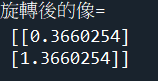程式碼內容 範例11-2：求 x=(2,3)，以原點逆時針旋轉90°，再施加水平向1剪力變換shear的像 3.範例11-2：求 x=(2,3)，以原點逆時針旋轉90°，再施加水平向1剪力變換shear的像 import numpy as np rad = np.radians(90) T1 = np.array([ [np.cos(rad),-np.sin(rad)], [np.sin(rad),np.cos(rad)] ]) T2 = np.array([ [1, 1], [0, 1] ]) x = np.array([ , ]) y = T2 @ T1 @ x print("兩次座標轉換後的像=\n", y) 成果圖示：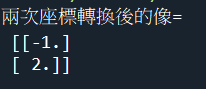程式碼內容 範例11-3：求點 (3,4,5) 對xz平面鏡射後的像 4.範例11-3：求點 (3,4,5) 對xz平面鏡射後的像 import numpy as np T1 = np.array([ [1,0,0], [0,-1,0], [0,0,1] ]) x = np.array([ ,, ]) y = T1 @ x print("對xz平面鏡射後的像\n", y) 成果圖示：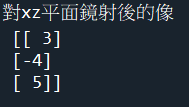程式碼內容 範例11-4：求點(-3,1)對x軸的正交投影 5.範例11-4：求點(-3,1)對x軸的正交投影 import numpy as np T1 = np.array([ [1,0], [0,0] ]) x = np.array([ [-3], ]) y = T1 @ x print('對x軸的正交投影=\n', y) 成果圖示：程式碼內容 範例11-5：求點(-2,1,2)，以y軸為中心旋轉45°旋轉後的像 6.範例11-5：求點(-2,1,2)，以y軸為中心旋轉45°旋轉後的像 import numpy as np rad = np.radians(45) T1 = np.array([ [np.cos(rad),0,np.sin(rad)], [0,1,0], [-np.sin(rad), 0, np.cos(rad)] ]) x = np.array([ [-2],, ]) y = T1 @ x print('以y軸為中心旋轉45°旋轉後的像=\n', y) 成果圖示：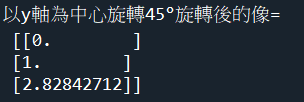程式碼內容
 chp12.簡化矩陣為echelon form梯形矩陣 1.python的sympy模組才有提供把矩陣簡化為echelon form梯形矩陣 2.sympy的線性代數指令 範例12-1：高斯喬丹消去法最後化簡的梯形矩陣 範例12-2：高斯喬丹消去法最後化簡的梯形矩陣 範例12-3：高斯喬丹消去法最後化簡的梯形矩陣 範例12-4：高斯喬丹消去法最後化簡的梯形矩陣 範例12-5：高斯喬丹消去法最後化簡的梯形矩陣 1.python的sympy模組才有提供把矩陣簡化為echelon form梯形矩陣 1.python的sympy模組才有提供把矩陣簡化為echelon form梯形矩陣 有兩種指令與方法 (1).指令：M.echelon_form() from sympy import * M = Matrix([ [1,1,2,9], [2,4,-3,1], [3,6,-5,0] ]) print('echelon form =\n', M.echelon_form()) (2).指令： from sympy import * M = Matrix([ [1,1,2,9], [2,4,-3,1], [3,6,-5,0] ]) print('echelon form =\n', M.rref()) (3).numpy模組沒有類似的指令 2.sympy的線性代數指令 2.sympy的線性代數指令 https://docs.sympy.org/latest/tutorial/matrices.html sympy線性代數完整教學： http://www.cfm.brown.edu/people/dobrush/cs52/sympy/ 3.範例12-1：高斯喬丹消去法最後化簡的梯形矩陣 3.範例12-1：高斯喬丹消去法最後化簡的梯形矩陣 #指令：M.rref() from sympy import * M = Matrix([ [1,1,2,9], [2,4,-3,1], [3,6,-5,0] ]) M_echelon = M.echelon_form() print('簡化的梯形矩陣的echelon form =\n', M_echelon) M_simple = M.rref() print('簡化的梯形矩陣的echelon form =\n', M_simple) print(M_simple.col(0)) print(M_simple.col(1)) print(M_simple.col(2)) 成果圖示：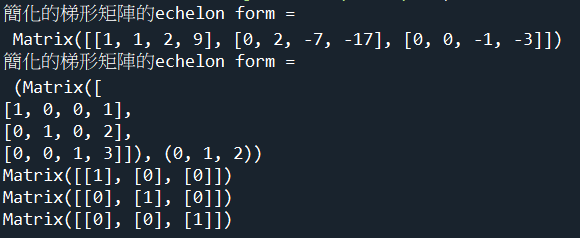程式碼內容 範例12-2：高斯喬丹消去法最後化簡的梯形矩陣 4.範例12-2：高斯喬丹消去法最後化簡的梯形矩陣 #指令：M.rref() #3x+y=1 #X+2y=0 from sympy import * M = Matrix([ [3,1,1], [1,2,0] ]) M_simple = M.rref() print('簡化的梯形矩陣的echelon form =\n', M_simple) 成果圖示：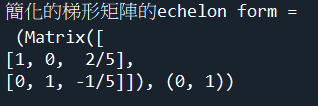程式碼內容 範例12-3：高斯喬丹消去法最後化簡的梯形矩陣 5.範例12-3：高斯喬丹消去法最後化簡的梯形矩陣 #指令：M.rref() from sympy import * M = Matrix([ [1,3,-2,0,2,0,0], [2,6,-5,-2,4,-3,-1], [0,0,5,10,0,15,5], [2,6,0,8,4,18,6] ]) M_simple = M.rref() print('簡化的梯形矩陣的echelon form =\n', M_simple) 成果圖示：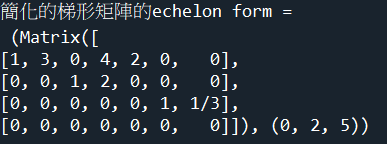程式碼內容 範例12-4：高斯喬丹消去法最後化簡的梯形矩陣 6.範例12-4：高斯喬丹消去法最後化簡的梯形矩陣 #指令：M.rref() from sympy import * M = Matrix([ [1,2,-3,6], [2,-1,4,1], [1,-1,1,3] ]) M_simple = M.rref() print('簡化的梯形矩陣的echelon form =\n', M_simple) 成果圖示：程式碼內容 範例12-5：高斯喬丹消去法最後化簡的梯形矩陣 7.範例12-5：高斯喬丹消去法最後化簡的梯形矩陣 #指令：M.rref() from sympy import * M = Matrix([ [-1,1,2,2], [3,-1,1,6], [-1,3,4,4] ]) M_simple = M.rref() print('簡化的梯形矩陣的echelon form =\n', M_simple) 成果圖示：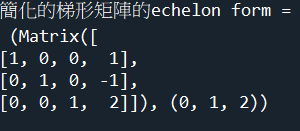程式碼內容
 chp13.像空間，核空間，rank，nullity 1.只有sympy可以解出核空間（null space) 2.範例13-1：像空間，核空間，rank，nullity 3.範例13-2：像空間，核空間，rank，nullity 4.範例13-3：像空間，核空間，rank，nullity 1.只有sympy可以解出核空間（null space) 1.只有sympy可以解出核空間（null space) 核空間向量集合 = null space = M.nullspace() 輸入空間維度 = input dimension of M= M.shape 輸出空間維度 = rank(M) = M.rank() 核空間維度 = 被轉換壓縮的空間維度 = nullity =M.shape - M.rank() 前的日期 2.範例13-1：像空間，核空間，rank，nullity 2.範例13-1：像空間，核空間，rank，nullity from sympy import * M = Matrix([ [3,1], [1,2] ]) M_nullsapce = M.nullspace() M_columnspace = M.columnspace() M_rank = M.rank() M_dim = M.shape M_nullity = M_dim - M_rank print('線性轉換後有壓縮空間 = 核空間向量集合 = null space=\n', M_nullsapce) print('輸出空間的向量之線性組和= column space= \n', M_columnspace) print('輸入空間維度 = input dimension of M=', M_dim) print('輸出空間維度 = rank(M)=', M_rank) print('核空間維度 = 被轉換壓縮的空間維度 = nullity=', M_nullity) 成果圖示：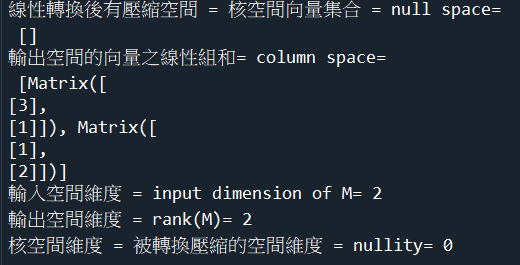程式碼內容 3.範例13-2：像空間，核空間，rank，nullity 3.範例13-2：像空間，核空間，rank，nullity from sympy import * M = Matrix([ [3,6], [1,2] ]) M_nullsapce = M.nullspace() M_columnspace = M.columnspace() M_rank = M.rank() M_dim = M.shape M_nullity = M_dim - M_rank print('線性轉換後有壓縮空間 = 核空間向量集合 = null space=\n', M_nullsapce) print('輸出空間的向量之線性組和= column space= \n', M_columnspace) print('輸入空間維度 = input dimension of M=', M_dim) print('輸出空間維度 = rank(M)=', M_rank) print('核空間維度 = 被轉換壓縮的空間維度 = nullity=', M_nullity) 成果圖示：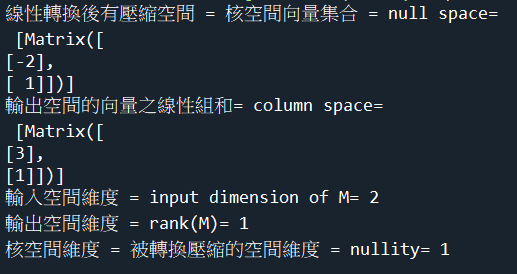程式碼內容 4.範例13-3：像空間，核空間，rank，nullity 4.範例13-3：像空間，核空間，rank，nullity from sympy import * M = Matrix([ [1,0], [0,1], [0,0] ]) M_nullsapce = M.nullspace() M_columnspace = M.columnspace() M_rank = M.rank() M_dim = M.shape M_nullity = M_dim - M_rank print('線性轉換後有壓縮空間 = 核空間向量集合 = null space=\n', M_nullsapce) print('輸出空間的向量之線性組和= column space= \n', M_columnspace) print('輸入空間維度 = input dimension of M=', M_dim) print('輸出空間維度 = rank(M)=', M_rank) print('核空間維度 = 被轉換壓縮的空間維度 = nullity=', M_nullity) 成果圖示：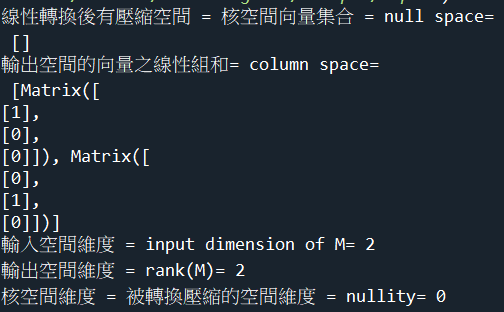程式碼內容 範例13-4:傳回自1970年1月1日凌晨0：0：0開始至今的秒數 5.範例13-4：像空間，核空間，rank，nullity from sympy import * M = Matrix([ [1,0,3,1], [0,1,1,2] ]) M_nullsapce = M.nullspace() M_columnspace = M.columnspace() M_rank = M.rank() M_dim = M.shape M_nullity = M_dim - M_rank print('線性轉換後有壓縮空間 = 核空間向量集合 = null space=\n', M_nullsapce) print('輸出空間的向量之線性組和= column space= \n', M_columnspace) print('輸入空間維度 = input dimension of M=', M_dim) print('輸出空間維度 = rank(M)=', M_rank) print('核空間維度 = 被轉換壓縮的空間維度 = nullity=', M_nullity) 成果圖示：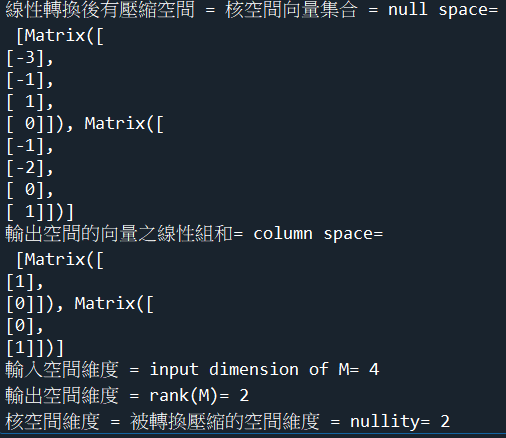程式碼內容
 chp14.線性系統有無限多組解的通解=特解＋齊次解 1.線性系統有無限多組解的通解=特解＋齊次解 2.求解線性系統，numpy與sympy指令的優缺點 3.計算矩陣的解空間的基底 範例14-1：求線性系統的解 範例14-2：無限多解系統的通解 範例14-3：求矩陣核空間的基底 範例14-4：求矩陣核空間的基底 範例14-5：求矩陣列空間的基底 範例14-6：求四個列向量所生產列空間的基底 範例14-7：求四個『行向量』所生產行空間的基底 範例14-8：證明A 的核空間和 A 的列空間彼此正交(垂直)p 1.線性系統有無限多組解的通解=特解＋齊次解 1.線性系統有無限多組解的通解=特解＋齊次解 (1).線性系統有無限多組解的完整解(通解) = 系統特解＋齊次解 = x0常數項 ＋ 壓縮後降階的解空間 =(Xx=0)的降階核空間之解，平移x0係數 (2).特解：Ax = b x0 為線性系統 Ax = b 之特解 ☎指令： (3).齊次解：Ax = 0 W 為滿足齊次系統 Ax = 0的齊次解集合 ☎指令：M.nullspace() (4).矩陣A的線性系統的完整解(通解）= x0 + W 2.求解線性系統，numpy與sympy指令的優缺點 2.求解線性系統，numpy與sympy指令的優缺點 （1）sympy模組 指令：solve_linear_system(M, x1,x2) 注意：M矩陣的最右邊=y=轉換後座標 from sympy import * x1,x2 = symbols('x1 x2') M = Matrix([ [1,1,4], [3,3,6] ]) ans = solve_linear_system(M, x1,x2) 優點1：可以自動處理唯一解，無解，無限多就解，不需要人工再去判別處理 優點2：無解，則自動顯示None 優點3：無限多解，則自動顯示通解（帶有x變數的通解） （2）numpy模組 指令：np.linalg.solve(A, Y) import numpy as np A = np.array([ [4,-2], [16,-8] ]) Y = np.array([ , ]) X = np.linalg.solve(A, Y) 缺點1：只能處理唯一解，無法自動處理無解，無限多就解，需要人工再去判別處理 缺點2：若是無解，程式會出現錯誤訊息 缺點3：若是無限多組解，程式會出現錯誤訊息 缺點4：必須用行列式det(M)，或是rank（M)，人工方式判別分類，來處理『唯一解，無解，無限多解』 （3）結論：若要解線性系統，只能用sympy來求解 不要用numpy模組求解，因為無法處理『無解，無限多組的通解』 3.計算矩陣的解空間的基底 3.計算矩陣的解空間的基底 有兩種： (1).計算矩陣的『列』空間或行空間的基底 ☎指令： M.nullspace() (2).計算矩陣的『行』空間或行空間的基底 ☎指令：M.columnspace() 範例14-1：求線性系統的解(比較sympy模組，numpy模組的解法) 4.範例14-1：求線性系統的解(比較sympy模組，numpy模組的解法) # -1x1+3x2+2x3 = 1 # x1+2x2-3x3 = -9 # 2x1+1x2-2x3 = -3 from sympy import * x1,x2,x3 = symbols('x1 x2 x3') M = Matrix([ [-1,3,2,1], [1,2,-3,-9], [2,1,-2,-3] ]) ans = solve_linear_system(M, x1, x2, x3) print('用sympy解聯立方程式 = ', ans) import numpy as np A = np.array([ [-1,3,2], [1,2,-3], [2,1,-2] ]) Y = np.array([ ,[-9],[-3] ]) X = np.linalg.solve(A, Y) print('用numpy解聯立方程式，X=\n', X) 成果圖示：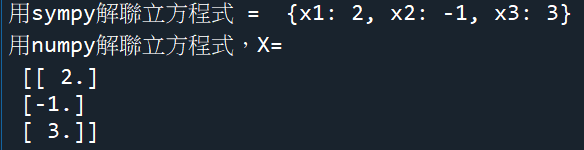程式碼內容 範例14-2：無限多解系統的通解（齊次特解x0+非齊次解xh） 5.範例14-2：無限多解系統的通解（齊次特解x0+非齊次解xh） #比較sympy模組，numpy模組的解法) #（1）sympy求解 from sympy import * x1,x2,x3,x4,x5,x6 = symbols('x1 x2 x3 x4 x5 x6') #注意：M矩陣的最右邊=y=轉換後座標 M = Matrix([ [1,3,-2,0,2,0,0], [2,6,-5,-2,4,-3,-1], [0,0,5,10,0,15,5], [2,6,0,8,4,18,6] ]) M_simple = M.rref() print('顯示高斯消去法簡化的梯形矩陣的echelon form =\n', M_simple) ans = solve_linear_system(M, x1,x2,x3,x4,x5,x6) print('用sympy解聯立方程式 = ', ans) M = Matrix([ [1,3,-2,0,2,0], [2,6,-5,-2,4,-3], [0,0,5,10,0,15], [2,6,0,8,4,18] ]) M_rank = M.rank() M_dim = M.shape M_nullity = M_dim - M_rank print('輸入空間維度 = input dimension of M=', M_dim) print('輸出空間維度 = rank(M)=', M_rank) print('核空間維度 = 被轉換壓縮的空間維度 = nullity=', M_nullity) M_nullsapce = M.nullspace() print('線性轉換後有壓縮空間 = 核空間向量集合 = null space=\n', M_nullsapce) #（2）numpy求解 import numpy as np A = np.array([ [1,3,-2,0,2,0], [2,6,-5,-2,4,-3], [0,0,5,10,0,15], [2,6,0,8,4,18] ]) Y = np.array([ ,[-1],, ]) print('用numpy解聯立方程式，X=（無法處理無限多組解）') X = np.linalg.solve(A, Y) print(X) 結論： （1）可以發現，無限多組解的通解=齊次項通解（(Ax=0)的null space)＋常數項係數x0) （2）常數項係數x0的算法有兩種： 1.高斯消去法，最後得到的常數值（例如，本題為x6=1/3) 2.null sapce的通解(Ax=0)裡面的所有變數=0代入後的值（本題目為x1~x5=0) 成果圖示：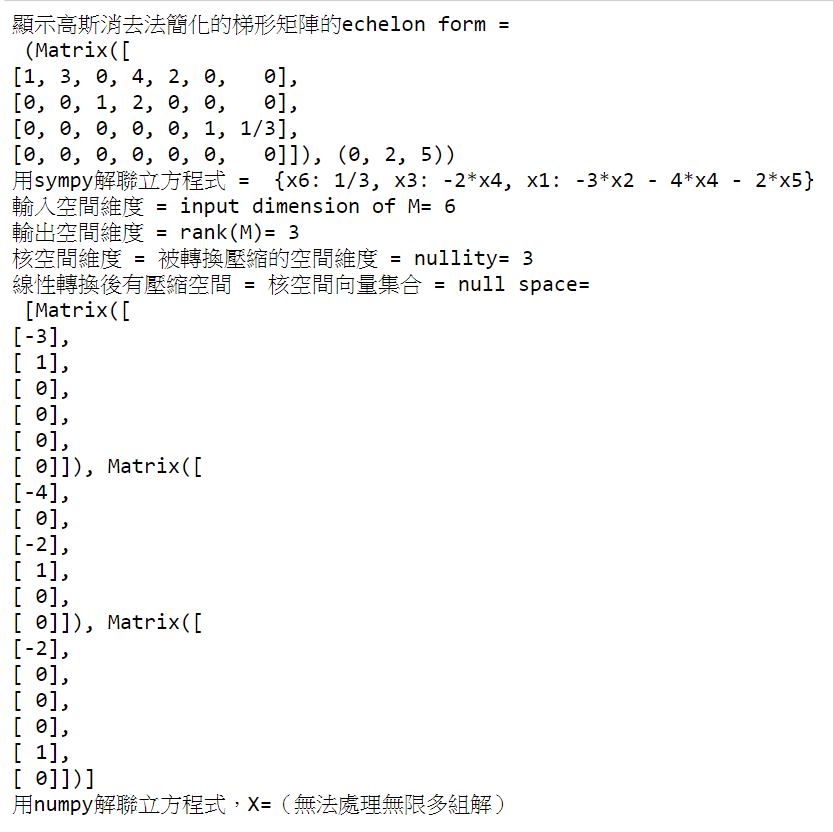程式碼內容 範例14-3：求矩陣核空間的基底 6.範例14-3：求矩陣核空間的基底 #（1）sympy求解 from sympy import * M = Matrix([ [1,3,-2,0,2,0], [2,6,-5,-2,4,-3], [0,0,5,10,0,15], [2,6,0,8,4,18] ]) M_simple = M.rref() print('顯示高斯消去法簡化的梯形矩陣的echelon form =\n', M_simple) M_rank = M.rank() M_dim = M.shape M_nullity = M_dim - M_rank print('輸入空間維度 = input dimension of M=', M_dim) print('輸出空間維度 = rank(M)=', M_rank) print('核空間維度 = 被轉換壓縮的空間維度 = nullity=', M_nullity) M_nullsapce = M.nullspace() M_columnspace = M.columnspace() print('線性轉換後有壓縮空間 = 核空間向量集合 = null space=\n', M_nullsapce) print('輸出行空間的向量之線性組和= column space= \n', M_columnspace) 成果圖示：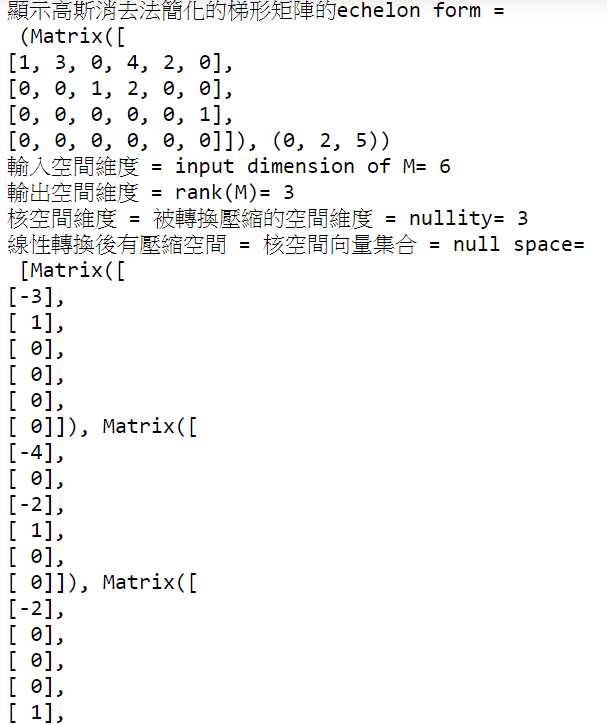程式碼內容 範例14-4：求矩陣核空間的基底 7.範例14-4：求矩陣核空間的基底 #（1）sympy求解 from sympy import * M = Matrix([ [1,-2,5,0,3], [0,1,3,0,0], [0,0,0,1,3], [0,0,0,0,0] ]) M_simple = M.rref() print('顯示高斯消去法簡化的梯形矩陣的echelon form =\n', M_simple) M_rowspace = M.rowspace() M_columnspace = M.columnspace() print('輸出列空間的向量之線性組和= row space= \n', M_rowspace) print('輸出行空間的向量之線性組和= column space= \n', M_columnspace) M_rank = M.rank() M_dim = M.shape M_nullity = M_dim - M_rank print('輸入空間維度 = input dimension of M=', M_dim) print('輸出空間維度 = rank(M)=', M_rank) print('核空間維度 = 被轉換壓縮的空間維度 = nullity=', M_nullity) M_nullsapce = M.nullspace() print('線性轉換後有壓縮空間 = 核空間向量集合 = null space=\n', M_nullsapce) 成果圖示：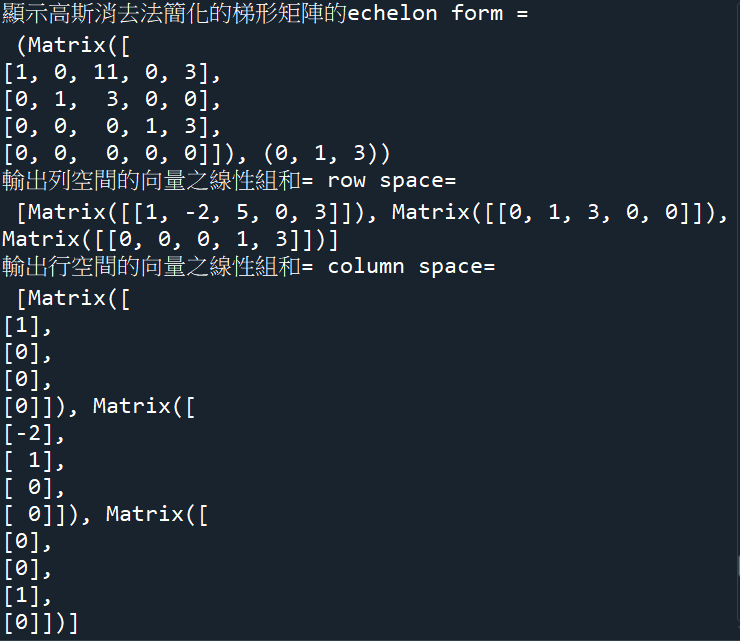程式碼內容 範例14-5：求矩陣列空間的基底 8.範例14-5：求矩陣列空間的基底 from sympy import * M = Matrix([ [1,-3,4,-2,5,4], [2,-6,9,-1,8,2], [2,-6,9,-1,9,7], [-1,3,-4,2,-5,-4] ]) M_simple = M.rref() print('顯示高斯消去法簡化的梯形矩陣的echelon form =\n', M_simple) M_rowspace = M.rowspace() M_columnspace = M.columnspace() print('輸出列空間的向量之線性組和= row space= \n', M_rowspace) print('輸出行空間的向量之線性組和= column space= \n', M_columnspace) 成果圖示：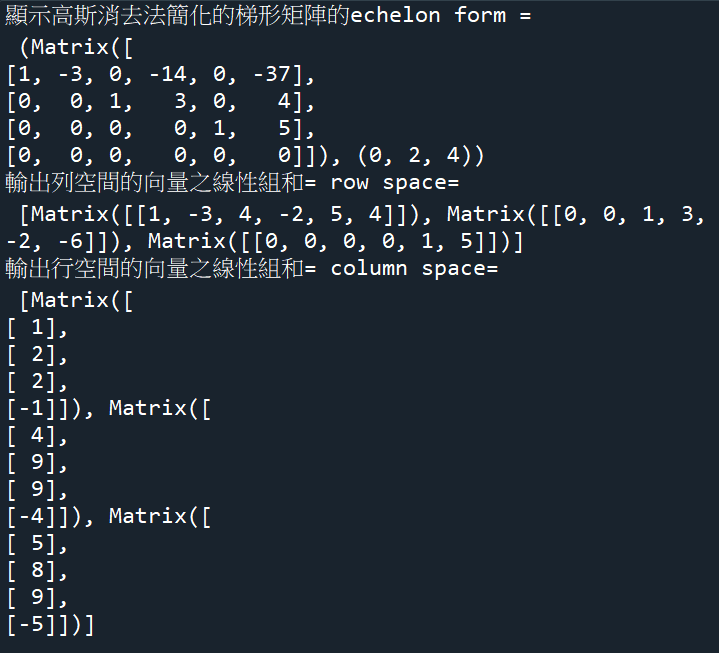程式碼內容 範例14-6：求四個列向量所生產列空間的基底 9.範例14-6：求四個列向量所生產列空間的基底 from sympy import * # v1=(1,-2,0,0,3) # v2=(2,-5,-3,-2,6) # v3=(0,5,15,10,0) # v4=(2,6,18,8,6) M = Matrix([ [1,-2,0,0,3], [2,-5,-3,-2,6], [0,5,15,10,0], [2,6,18,8,6] ]) M_simple = M.rref() print('顯示高斯消去法簡化的梯形矩陣的echelon form =\n', M_simple) M_rowspace = M.rowspace() M_columnspace = M.columnspace() print('輸出列空間的向量之線性組和= row space= \n', M_rowspace) print('輸出行空間的向量之線性組和= column space= \n', M_columnspace) 成果圖示：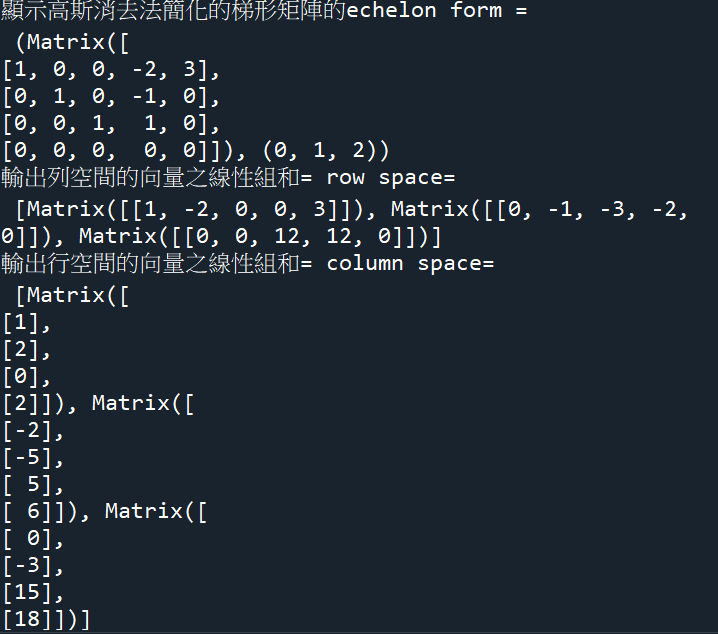程式碼內容 範例14-7：求四個『行向量』所生產行空間的基底 10.範例14-7：求四個『行向量』所生產行空間的基底 from sympy import * # v1=(1,-2,0,3) # v2=(2,-5,-3,6) # v3=(0,1,3,0) # v4=(2,-1,4,-7) # v5=(5,-8,1,2) M = Matrix([ [1,2,0,2,5], [-2,-5,1,-1,-8], [0,-3,3,4,1], [3,6,0,-7,2] ]) M_simple = M.rref() print('顯示高斯消去法簡化的梯形矩陣的echelon form =\n', M_simple) M_rowspace = M.rowspace() M_columnspace = M.columnspace() print('輸出列空間的向量之線性組和= row space= \n', M_rowspace) print('輸出行空間的向量之線性組和= column space= \n', M_columnspace) 成果圖示：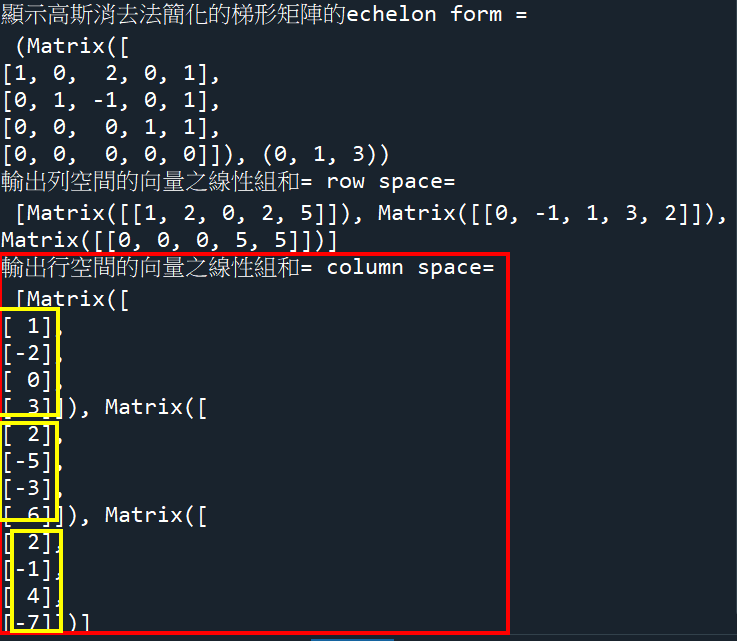程式碼內容 範例14-8：證明A 的核空間和 A 的列空間彼此正交(垂直)p 11.範例14-8：證明A 的核空間和 A 的列空間彼此正交(垂直) from sympy import * M = Matrix([ [1,3,-2,0,2,0], [2,6,-5,-2,4,-3], [0,0,5,10,0,15], [2,6,0,8,4,18] ]) M_nullsapce = M.nullspace() M_rowspace = M.rowspace() print('線性轉換後有壓縮空間 = 核空間向量集合 = null space=\n', M_nullsapce) print('輸出列空間的向量之線性組和= column space= \n', M_rowspace) n1, n2, n3 = M.nullspace() r1, r2, r3 = M.rowspace() print('n1 = ', n1) print('r1 = ', r1) print('n1.dot(r1)=核空間和列空間彼此正交(內積=0) = ', n1.dot(r1)) 成果圖示：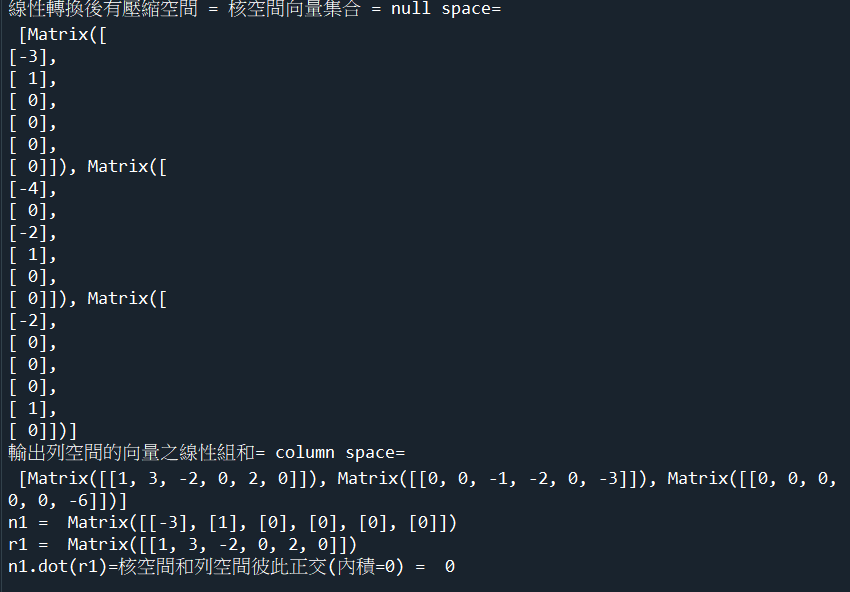程式碼內容
 chp15.算出矩陣向量的垂直正則基底向量(長度=1) 1.如何建立單範正交基底 2.sympy的指令 範例15-1：使用葛蘭-史密特正交程序，把矩陣向量，製造出單範正交基底 範例15-2：使用QR分解法，把矩陣向量，製造出單範正交基底 1.如何建立單範正交基底 1.如何建立單範正交基底 (1).方法：葛蘭-史密特正交程序 gram Schmidt process 功能：可以把非正交非單範的基底，製造出『單範正交基底向量』 (2).方法：QR分解法 要先進行葛蘭-史密特正交程序運算，得出q1,q2,q3 可以把矩陣分解成QR Q的行空間，就是單範正交基底向量 範2.sympy的指令 2.sympy的指令 （1）使用G葛蘭-史密特法：Gram-Schmidt orthogonalization 指令：GramSchmidt(M) 指令：GramSchmidt(M, True) （2）QR分解法 指令：Q, R = A.QRdecomposition() （3）建議使用：QR分解法 優點1：matrix格式不用改變 優點2：直接說正則基底，不用再正規化 優點3：程式碼簡短 範例15-1：使用葛蘭-史密特正交程序，把矩陣向量，製造出單範正交基底 3.範例15-1：使用葛蘭-史密特正交程序，把矩陣向量，製造出單範正交基底 #指令：GramSchmidt(M) #缺點1：GramSchmidt(M)是正交基底，長度不為1，還需要再正規化，轉成正則基底 #改善：GramSchmidt(M, True)則會正規化，變成正則基底（長度=1） #缺點2：matrix輸入的格式與一般格式不同 from sympy import * #錯誤的矩陣格式 M = Matrix([ [1,0,0], [1,1,0], [1,1,1] ]) #注意：GramSchmidt(M)方法的矩陣，必須以行向量的方式輸入，外圍用[]list串列包圍 M = [Matrix([1,1,1]), Matrix([0,1,1]), Matrix([0,0,1])] #若要建立正交基底（沒有正規化）：M_GS = GramSchmidt(M, False)= GramSchmidt(M) #若要建立正則基底（有正規化）：M_GS = GramSchmidt(M, True) M_GS = GramSchmidt(M, True) q1,q2,q3 = GramSchmidt(M, True) print('1.把M矩陣製造出正則基底=\n', M_GS) print('q1正則基底 = ', q1) print('q2正則基底 = ', q2) print('q3正則基底 = ', q3) 成果圖示：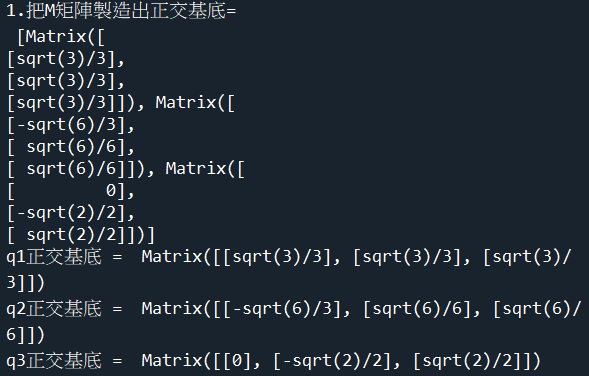程式碼內容 範例15-2：使用QR分解法，把矩陣向量，製造出單範正交基底 4.範例15-2：使用QR分解法，把矩陣向量，製造出單範正交基底 #指令：GramSchmidt(M) #優點：Q就是正則基底，長度為1，不需要再正規化了 from sympy import * M = Matrix([ [1,0,0], [1,1,0], [1,1,1] ]) Q,R = M.QRdecomposition() print("用QR分解法，求出正則基底=\n", Q) q1 = Q.col(0) q2 = Q.col(1) q3 = Q.col(2) print('q1 = ', q1) print('q2 = ', q2) print('q3 = ', q3) 成果圖示：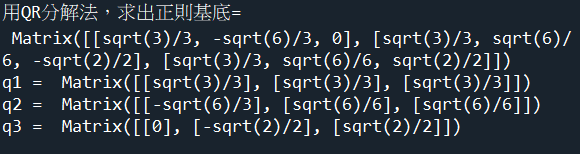程式碼內容